Lower Columbia River Fish Population Indexing 2019

The suggested citation for this analytic report is:

Thorley, J.L. (2020) Lower Columbia River Fish Population Indexing 2019. A Poisson Consulting Analysis Appendix. URL: https://www.poissonconsulting.ca/f/1566579922.

Background

In the mid 1990s BC Hydro began operating Hugh L. Keenleyside (HLK) Dam to reduce dewatering of Mountain Whitefish and Rainbow Trout eggs.

The primary goal of the Lower Columbia River Fish Population Indexing program is to answer two key management questions:

What are the abundance, growth rate, survival rate, body condition, age distribution, and spatial distribution of subadult and adult Whitefish, Rainbow Trout, and Walleye in the Lower Columbia River?

What is the effect of inter-annual variability in the Whitefish and Rainbow Trout flow regimes on the abundance, growth rate, survival rate, body condition, and spatial distribution of subadult and adult Whitefish, Rainbow Trout, and Walleye in the Lower Columbia River?

The inter-annual variability in the Whitefish and Rainbow Trout flow regimes was quantified in terms of the percent egg dewatering as greater flow variability is associated with more egg stranding.

Methods

Data Preparation

The fish indexing data were provided by Okanagan Nation Alliance and Golder Associates in the form of an Access database. The discharge and temperature data were obtained from the Columbia Basin Hydrological Database maintained by Poisson Consulting. The Rainbow Trout egg dewatering estimates were provided by CLBMON-46 and the Mountain Whitefish egg stranding estimates by Golder Associates (2013).

Discharge

Missing hourly discharge values for Hugh-Keenleyside Dam (HLK), Brilliant Dam (BRD) and Birchbank (BIR) were estimated by first leading the BIR values by 2 hours to account for the lag. Values missing at just one of the dams were then estimated assuming $$HLK + BRD = BIR$$. Negative values were set to be zero. Next, missing values spanning $$\leq$$ 28 days were estimated at HLK and BRD based on linear interpolation. Finally any remaining missing values at BIR were set to be $$HLK + BRD$$.

The data were prepared for analysis using R version 4.0.2 .

Data Analysis

Model parameters were estimated using hierarchical Bayesian methods. The parameters were produced using JAGS and STAN . For additional information on Bayesian estimation the reader is referred to .

The one exception is the length-at-age estimates which were produced using the mixdist R package which implements Maximum Likelihood with Expectation Maximization.

Unless indicated otherwise, the Bayesian analyses used normal and uniform prior distributions that were vague in the sense that they did not constrain the posteriors . The posterior distributions were estimated from 1500 Markov Chain Monte Carlo (MCMC) samples thinned from the second halves of 3 chains . Model convergence was confirmed by ensuring that $$\hat{R} \leq 1.05$$ and $$\textrm{ESS} \geq 150$$ for each of the monitored parameters . Where $$\hat{R}$$ is the potential scale reduction factor and $$\textrm{ESS}$$ is the effective sample size .

The parameters are summarised in terms of the point estimate, standard deviation (sd), the z-score, lower and upper 95% confidence/credible limits (CLs) and the p-value . For ML models, the point estimate is the MLE, the standard deviation is the standard error, the z-score is $$\mathrm{MLE}/\mathrm{sd}$$ and the 95% CLs are the $$\mathrm{MLE} \pm 1.96 \cdot \mathrm{sd}$$. For Bayesian models, the estimate is the median (50th percentile) of the MCMC samples, the z-score is $$\mathrm{mean}/\mathrm{sd}$$ and the 95% CLs are the 2.5th and 97.5th percentiles. A p-value of 0.05 indicates that the lower or upper 95% CL is 0.

Where relevant, model adequacy was confirmed by examination of residual plots for the full model(s).

The results are displayed graphically by plotting the modeled relationships between particular variables and the response(s) with the remaining variables held constant. In general, continuous and discrete fixed variables are held constant at their mean and first level values, respectively, while random variables are held constant at their typical values (expected values of the underlying hyperdistributions) . When informative the influence of particular variables is expressed in terms of the effect size (i.e., percent change in the response variable) with 95% confidence/credible intervals .

The analyses were implemented using R version 4.0.2 and the mbr family of packages.

Model Descriptions

Condition

The expected weight of fish of a given length were estimated from the data using an allometric mass-length model .

$W = \alpha L^{\beta}$

Key assumptions of the condition model include:

• The expected weight is allowed to vary with length and date.
• The expected weight is allowed to vary randomly with year.
• The relationship between weight and length is allowed to vary with date.
• The relationship between weight and length is allowed to vary randomly with year.
• The residual variation in weight is log-normally distributed.

Only previously untagged fish were included in models to avoid potential effects of tagging on body condition. Preliminary analyses indicated that the annual variation in weight was not correlated with the annual variation in the relationship between weight and length.

Growth

Annual growth of fish were estimated from the inter-annual recaptures using the Fabens method for estimating the von Bertalanffy growth curve . This curve is based on the premise that:

$\frac{\text{d}L}{\text{d}t} = k (L_{\infty} - L)$

where $$L$$ is the length of the individual, $$k$$ is the growth coefficient and $$L_{\infty}$$ is the maximum length.

Integrating the above equation gives:

$L_t = L_{\infty} (1 - e^{-k(t - t_0)})$

where $$L_t$$ is the length at time $$t$$ and $$t_0$$ is the time at which the individual would have had zero length.

The Fabens form allows

$L_r = L_c + (L_{\infty} - L_c) (1 - e^{-kT})$

where $$L_r$$ is the length at recapture, $$L_c$$ is the length at capture and $$T$$ is the time between capture and recapture.

Key assumptions of the growth model include:

• The mean maximum length $$L_{\infty}$$ is constant.
• The growth coefficient $$k$$ is allowed to vary randomly with year.
• The residual variation in growth is normally distributed.

The growth model was only fitted to Walleye with a fork length at release less than 450 mm.

Movement

The extent to which sites are closed, i.e., fish remain at the same site between sessions, was evaluated with a logistic ANCOVA . The model estimates the probability that intra-annual recaptures were caught at the same site versus a different one. Key assumptions of the site fidelity model include:

• The expected site fidelity is allowed to vary with fish length.
• Observed site fidelity is Bernoulli distributed.

Length as a second-order polynomial was not found to be a significant predictor for site fidelity.

The estimated probability of being caught at the same site versus a different site was then converted into the site fidelity by assuming that those fish which were recaught at a different site represented just 32 % of those that left the site. The correction factor corresponds to the proportion of the river bank that belongs to index sites.

Length-At-Age

The expected length-at-age of Mountain Whitefish and Rainbow Trout were estimated from annual length-frequency distributions using a finite mixture distribution model

There were assumed to be three distinguishable normally-distributed age-classes for Mountain Whitefish (Age-0, Age-1, Age-2 and Age-3+) two for Rainbow Trout (Age-0, Age-1, Age-2+). Initially the model was fitted to the data from all years combined. The model was then fitted to the data for each year separately with the initial values set to be the estimates from the combined values. The only constraints were that the standard deviations of the MW age-classes were identical in the combined analysis and fixed at the initial values in the individual years.

Rainbow Trout and Mountain Whitefish were categorized as Fry (Age-0), Juvenile (Age-1) and Adult (Age-2+) based on their length-based ages. All Walleye were considered to be Adults.

Survival

The annual adult survival rate was estimated by fitting a Cormack-Jolly-Seber model to inter-annual recaptures of adults.

Key assumptions of the survival model include:

• Survival varies randomly with year.
• The encounter probability for adults is allowed to vary with the total bank length sampled.

Preliminary analysis indicated that only including visits to index sites did not substantially change the results.

Observer Length Correction

The annual bias (inaccuracy) and error (imprecision) in observer’s fish length estimates were quantified from the divergence of the length distribution of their observed fish from the length distribution of the measured fish. More specifically, the length correction that minimised the Jensen-Shannon divergence (Lin 1991) between the two distributions provided a measure of the inaccuracy while the minimum divergence (the Jensen-Shannon divergence was calculated with log to base 2 which means it lies between 0 and 1) provided a measure of the imprecision.

Capture Efficiency

The probability of capture was estimated using a recapture-based binomial model .

Key assumptions of the capture efficiency model include:

• The capture probability varies randomly by session within year.
• The probability of a marked fish remaining at a site is the estimated site fidelity.
• The number of recaptures is described by a binomial distribution.

Abundance

The abundance was estimated from the catch and bias-corrected observer count data using an overdispersed Poisson model .

Key assumptions of the abundance model include:

• The fish density varies randomly with site, year and site within year.
• The capture efficiency at a typical fish density is the point estimate for a typical session from the capture efficiency model.
• The count efficiency varies from the capture efficiency.
• The capture efficiency (but not the count efficiency) varies with density.
• The overdispersion varies by visit type (count or catch).
• The catches and counts are described by a gamma-Poisson distribution.
Distribution

The distribution was calculated in terms of the Shannon index of evenness in each year for each species and life-stage. The index was calculated using the following formula where $$S$$ is the number of sites and $$p_i$$ is the proportion of the total density belonging to the ith site

$E = \frac{-\sum p_i \log(p_i)}{\log(S)}$

Survival (Abundance-based)

The subadult ($$S_t$$) and adult ($$A_t$$) abundance estimates were used to calculate the subadult and adult survival ($$\phi_t$$) in year $$t$$ based on the relationship

$\phi_t = \frac{A_t}{S_{t-1} + A_{t-1}}$

Weight

The weight ($$W_t$$) in year $$t$$ was estimated from the expected adult length using the condition model.

Fecundity

Mountain Whitefish

The fecundity-weight relationship for Mountain Whitefish was estimated from data collected by for the Madison River, Montana. The data were analysed using an allometric model of the form

$F = \alpha W^{\beta}$

Key assumptions of the fecundity model include:

• The residual variation in fecundity is log-normally distributed.
Rainbow Trout

Following the fecundity ($$F_t$$) in year $$t$$ of an adult female Rainbow Trout was calculated from the expected weight ($$W_t$$) in grams using the equation:

$F_t = 3.8 \cdot W_t^{0.9}$

Egg Deposition

The total egg deposition ($$E_t$$) in year $$t$$ was calculated according to the equation $E_t = F_t * \frac{A_t}{2}$

Stock-Recruitment

The relationship between the total number of eggs deposited ($$E_t$$) and the resultant number of subadults (age-1 recruits) ($$S_{t+1}$$) was estimated using a Beverton-Holt stock-recruitment model :

$S_{t+1} = \frac{\alpha \cdot E_t}{1 + \beta \cdot E_t}$

where $$\alpha$$ is the egg to age-1 survival at low density and $$\beta$$ is the density-dependence.

Key assumptions of the stock-recruitment model include:

• The egg to recruit survival at low density ($$\alpha$$) was likely less than 1% (the prior distribution for $$\alpha$$ was a zero truncated normal with standard deviation of 0.005.
• The residual variation in the number of recruits is log-normally distributed.

The expected egg survival for a given egg deposition is $$S / E_t$$ which is given by the equation

$\phi_E = \frac{\alpha}{1 + \beta * E}$

Age-Ratios

The proportion of Age-1 Mountain Whitefish $$r^1_t$$ from a given spawn year $$t$$ is calculated from the relative abundance of Age-1 & Age-2 fish $$N^1_t$$ & $$N^2_t$$ respectively, which were lead or lagged so that all values were with respect to the spawn year:

$r^1_t = \frac{N^1_{t+2}}{N^1_{t+2} + N^2_{t+2}}$

The relative abundances of Age-1 and Age-2 fish were taken from the proportions of each age-class in the length-at-age analysis.

As the number of Age-2 fish might be expected to be influenced by the percentage egg loss $$Q_t$$ three years prior, the predictor variable $$\Pi_t$$ used is:

$\Pi_t = \textrm{log}(Q_t/Q_{t-1})$

The ratio was logged to ensure it was symmetrical about zero .

The relationship between $$r^1_t$$ and $$\Pi_t$$ was estimated using a Bayesian regression loss model.

Key assumptions of the final model include:

• The log odds of the proportion of Age-1 fish varies with the log of the ratio of the percent egg losses.
• The residual variation is normally distributed.

The relationship between percent dewatering and subsequent recruitment is expected to depend on stock abundance which might be changing over the course of the study. Consequently, preliminary analyses allowed the slope of the regression line to change by year. However, year was not a significant predictor and was therefore removed from the final model. The effect of dewatering on Mountain Whitefish abundance was expressed in terms of the predicted percent change in Age-1 Mountain Whitefish abundance by egg loss in the spawn year relative to 10% egg loss in the spawn year. The egg loss in the previous year was fixed at 10%. The percent change could not be calculated relative to 0% in the spawn or previous year as $$\Pi_t$$ is undefined in either case.

Model Templates

Condition

data {
int nYear;
int nObs;

vector[nObs] Length;
vector[nObs] Weight;
vector[nObs] Dayte;
int Year[nObs];

parameters {
real bWeight;
real bWeightLength;
real bWeightDayte;
real bWeightLengthDayte;
real sWeightYear;
real sWeightLengthYear;

vector[nYear] bWeightYear;
vector[nYear] bWeightLengthYear;
real sWeight;

model {

vector[nObs] eWeight;

bWeight ~ normal(5, 5);
bWeightLength ~ normal(3, 2);

bWeightDayte ~ normal(0, 2);
bWeightLengthDayte ~ normal(0, 2);

sWeightYear ~ normal(0, 2);
sWeightLengthYear ~ normal(0, 2);

for (i in 1:nYear) {
bWeightYear[i] ~ normal(0, exp(sWeightYear));
bWeightLengthYear[i] ~ normal(0, exp(sWeightLengthYear));
}

sWeight ~ normal(0, 5);
for(i in 1:nObs) {
eWeight[i] = bWeight + bWeightDayte * Dayte[i] + bWeightYear[Year[i]] + (bWeightLength + bWeightLengthDayte * Dayte[i] + bWeightLengthYear[Year[i]]) * Length[i];
Weight[i] ~ lognormal(eWeight[i], exp(sWeight));
}

Block 1.

Growth

.model {
bK ~ dnorm (0, 5^-2)
sKYear ~ dnorm(0, 5^-2)

for (i in 1:nYear) {
bKYear[i] ~ dnorm(0, exp(sKYear)^-2)
log(eK[i]) <- bK + bKYear[i]
}

bLinf ~ dnorm(500, 250^-2) T(100, 1000)
sGrowth ~ dnorm(0, 5^-2)
for (i in 1:length(Year)) {
eGrowth[i] <- (bLinf - LengthAtRelease[i]) * (1 - exp(-sum(eK[Year[i]:(Year[i] + dYears[i] - 1)])))
Growth[i] ~ dnorm(max(eGrowth[i], 0), exp(sGrowth)^-2)
}

Block 2.

Movement

.model {

bFidelity ~ dnorm(0, 2^-2)
bLength ~ dnorm(0, 2^-2)

for (i in 1:length(Fidelity)) {
logit(eFidelity[i]) <- bFidelity + bLength * Length[i]
Fidelity[i] ~ dbern(eFidelity[i])
}

Block 3.

Survival

.model{
bEfficiency ~ dnorm(0, 5^-2)
bEfficiencySampledLength ~ dnorm(0, 5^-2)

bSurvival ~ dnorm(0, 5^-2)

sSurvivalYear ~ dnorm(0, 5^-2)
for(i in 1:nYear) {
bSurvivalYear[i] ~ dnorm(0, exp(sSurvivalYear)^-2)
}

for(i in 1:(nYear-1)) {
logit(eEfficiency[i]) <- bEfficiency + bEfficiencySampledLength * SampledLength[i]
logit(eSurvival[i]) <- bSurvival + bSurvivalYear[i]

eProbability[i,i] <- eSurvival[i] * eEfficiency[i]
for(j in (i+1):(nYear-1)) {
eProbability[i,j] <- prod(eSurvival[i:j]) * prod(1-eEfficiency[i:(j-1)]) * eEfficiency[j]
}
for(j in 1:(i-1)) {
eProbability[i,j] <- 0
}
}
for(i in 1:(nYear-1)) {
eProbability[i,nYear] <- 1 - sum(eProbability[i,1:(nYear-1)])
}

for(i in 1:(nYear - 1)) {
Marray[i, 1:nYear] ~ dmulti(eProbability[i,], Released[i])
}

Block 4.

Capture Efficiency

.model {

bEfficiency ~ dnorm(-4, 3^-2)

sEfficiencySessionAnnual ~ dnorm(0, 2^-2) T(0,)
for (i in 1:nSession) {
for (j in 1:nAnnual) {
bEfficiencySessionAnnual[i, j] ~ dnorm(0, sEfficiencySessionAnnual^-2)
}
}

for (i in 1:length(Recaptures)) {

logit(eEfficiency[i]) <- bEfficiency + bEfficiencySessionAnnual[Session[i], Annual[i]]

eFidelity[i] ~ dnorm(Fidelity[i], FidelitySD[i]^-2) T(FidelityLower[i], FidelityUpper[i])
Recaptures[i] ~ dbin(eEfficiency[i] * eFidelity[i], Tagged[i])
}

Block 5.

Abundance

.model {
bDensity ~ dnorm(5, 5^-2)

sDensityAnnual ~ dnorm(0, 2^-2)
for (i in 1:nAnnual) {
bDensityAnnual[i] ~ dnorm(0, exp(sDensityAnnual)^-2)
}

sDensitySite ~ dnorm(0, 2^-2)
sDensitySiteAnnual ~ dnorm(0, 2^-2)
for (i in 1:nSite) {
bDensitySite[i] ~ dnorm(0, exp(sDensitySite)^-2)
for (j in 1:nAnnual) {
bDensitySiteAnnual[i, j] ~ dnorm(0, exp(sDensitySiteAnnual)^-2)
}
}

bEfficiencyVisitType <- 0
bEfficiencyVisitTypeDensity ~ dnorm(0, 2^-2)
for (i in 2:nVisitType) {
bEfficiencyVisitType[i] ~ dnorm(0, 2^-2)
bEfficiencyVisitTypeDensity[i] <- 0
}

sDispersion ~ dnorm(0, 2^-2)
sDispersionVisitType <- 0
for(i in 2:nVisitType) {
sDispersionVisitType[i] ~ dnorm(0, 2^-2)
}

for (i in 1:length(Fish)) {
log(eDensity[i]) <- bDensity + bDensitySite[Site[i]] + bDensityAnnual[Annual[i]] + bDensitySiteAnnual[Site[i],Annual[i]]

eAbundance[i] <- eDensity[i] * SiteLength[i]

logit(eEfficiency[i]) <- logit(Efficiency[i]) + bEfficiencyVisitType[VisitType[i]] + bEfficiencyVisitTypeDensity[VisitType[i]] * (eDensity[i] - exp(bDensity + sDensityAnnual^2/2 + sDensitySite^2/2 + sDensitySiteAnnual^2/2))

log(esDispersion[i]) <- sDispersion + sDispersionVisitType[VisitType[i]]

eDispersion[i] ~ dgamma(esDispersion[i]^-2 + 0.1, esDispersion[i]^-2 + 0.1)
eFish[i] <- eAbundance[i] * ProportionSampled[i] * eEfficiency[i]
Fish[i] ~ dpois(eFish[i] * eDispersion[i])
}

Block 6.

Fecundity

model {
bFecundity ~ dnorm(3, 2^-2) T(0,)
bFecundityWeight ~ dnorm(1, 1^-2) T(0,)

sFecundity ~ dnorm(0, 1^-2) T(0,)
for(i in 1:length(Weight)) {
eFecundity[i] = log(bFecundity) + bFecundityWeight * log(Weight[i])
Fecundity[i] ~ dlnorm(eFecundity[i], sFecundity^-2)
}

Block 7.

Stock-Recruitment

.model {
bAlpha ~ dnorm(0, 0.005^-2) T(0,)
bBeta ~ dnorm(0, 0.01^-2) T(0, )
bEggLoss ~ dnorm(0, 100^-2)

sRecruits ~ dnorm(0, 1^-2) T(0,)
for(i in 1:length(Recruits)){
log(eRecruits[i]) <- log(bAlpha * Eggs[i] / (1 + bBeta * Eggs[i])) + bEggLoss * EggLoss[i]
Recruits[i] ~ dlnorm(log(eRecruits[i]), sRecruits^-2)
}

Block 8.

Age-Ratios

.model{
bProbAge1 ~ dnorm(0, 2^-2)
bProbAge1Loss ~ dnorm(0, 2^-2)

sProbAge1 ~ dnorm(0, 2^-2) T(0,)
for(i in 1:length(Age1Prop)){
eAge1Prop[i] <- bProbAge1 + bProbAge1Loss * LossLogRatio[i]
Age1Prop[i] ~ dnorm(eAge1Prop[i], sProbAge1^-2)
}

Block 9.

Results

Tables

Condition

Table 1. Parameter descriptions.

Parameter Description
bWeight Intercept of log(eWeight)
bWeightDayte Effect of Dayte on bWeight
bWeightLength Intercept of effect of Length on bWeight
bWeightLengthDayte Effect of Dayte on bWeightLength
bWeightLengthYear[i] Effect of ith Year on bWeightLength
bWeightYear[i] Effect of ith Year on bWeight
Dayte[i] Standardised day of year ith fish was captured
eWeight[i] Expected Weight of ith fish
Length[i] Log-transformed and centered fork length of ith fish
sWeight Log standard deviation of residual variation in log(Weight)
sWeightLengthYear Log standard deviation of bWeightLengthYear
sWeightYear Log standard deviation of bWeightYear
Weight[i] Recorded weight of ith fish
Year[i] Year ith fish was captured
Mountain Whitefish

Table 2. Model coefficients.

term estimate sd zscore lower upper pvalue
bWeight 5.4584816 0.0094215 579.366814 5.4387979 5.4771345 0.0006662
bWeightDayte -0.0185924 0.0019068 -9.744552 -0.0222386 -0.0147221 0.0006662
bWeightLength 3.1611315 0.0235436 134.264923 3.1172191 3.2054775 0.0006662
bWeightLengthDayte -0.0138623 0.0047531 -2.920980 -0.0231713 -0.0044996 0.0073284
sWeight -1.9101787 0.0059346 -321.823757 -1.9209560 -1.8978873 0.0006662
sWeightLengthYear -2.2801420 0.1839999 -12.350844 -2.6126335 -1.9027586 0.0006662
sWeightYear -3.0853356 0.1651631 -18.669259 -3.3972819 -2.7517237 0.0006662

Table 3. Model summary.

n K nchains niters nthin ess rhat converged
14721 7 3 500 2 423 1.011 TRUE
Rainbow Trout

Table 4. Model coefficients.

term estimate sd zscore lower upper pvalue
bWeight 6.0085065 0.0058014 1035.72561 5.9974303 6.0207085 0.0006662
bWeightDayte -0.0043596 0.0012076 -3.61860 -0.0066671 -0.0019982 0.0006662
bWeightLength 2.9227486 0.0119517 244.56392 2.8995689 2.9459682 0.0006662
bWeightLengthDayte 0.0372528 0.0036358 10.26442 0.0305628 0.0447161 0.0006662
sWeight -2.2731632 0.0058706 -387.23188 -2.2852034 -2.2616154 0.0006662
sWeightLengthYear -2.9419511 0.1935919 -15.13472 -3.2913492 -2.5260907 0.0006662
sWeightYear -3.6380073 0.1656295 -21.93262 -3.9363094 -3.2807587 0.0006662

Table 5. Model summary.

n K nchains niters nthin ess rhat converged
15429 7 3 500 2 316 1.006 TRUE
Walleye

Table 6. Model coefficients.

term estimate sd zscore lower upper pvalue
bWeight 6.2882829 0.0076693 819.930784 6.2729275 6.3030276 0.0006662
bWeightDayte 0.0156879 0.0013761 11.435309 0.0129814 0.0182437 0.0006662
bWeightLength 3.2315414 0.0184538 175.112707 3.1957776 3.2662059 0.0006662
bWeightLengthDayte -0.0095241 0.0083553 -1.136963 -0.0265168 0.0065334 0.2445037
sWeight -2.3745891 0.0072884 -325.794383 -2.3892316 -2.3598153 0.0006662
sWeightLengthYear -2.5580662 0.1935659 -13.172976 -2.9168206 -2.1664570 0.0006662
sWeightYear -3.3536993 0.1609045 -20.810332 -3.6449256 -3.0057883 0.0006662

Table 7. Model summary.

n K nchains niters nthin ess rhat converged
9548 7 3 500 2 261 1.01 TRUE

Growth

Table 8. Parameter descriptions.

Parameter Description
bK Intercept of log(eK)
bKYear[i] Effect of ith Year on bK
bLinf Mean maximum length
dYears[i] Years between release and recapture of ith recapture
eGrowth Expected Growth between release and recapture
eK[i] Expected von Bertalanffy growth coefficient from i-1th to ith year
Growth[i] Observed growth between release and recapture of ith recapture
LengthAtRelease[i] Length at previous release of ith recapture
sGrowth Log standard deviation of residual variation in Growth
sKYear Log standard deviation of bKYear
Year[i] Release year of ith recapture
Mountain Whitefish

Table 9. Model coefficients.

term estimate sd zscore lower upper pvalue
bK -0.9298442 0.0983887 -9.482098 -1.132372 -0.7407841 0.0006662
bLinf 393.1783613 3.1119832 126.348086 387.250321 399.2876248 0.0006662
sGrowth 2.4530782 0.0444962 55.135395 2.362481 2.5407411 0.0006662
sKYear -1.1287979 0.2485045 -4.516800 -1.590515 -0.6117755 0.0006662

Table 10. Model summary.

n K nchains niters nthin ess rhat converged
268 4 3 500 20 674 1.005 TRUE
Rainbow Trout

Table 11. Model coefficients.

term estimate sd zscore lower upper pvalue
bK -0.1666498 0.0776961 -2.194483 -0.3235851 -0.0153518 0.0326449
bLinf 484.7735665 2.6074266 185.891683 479.6234263 489.9486010 0.0006662
sGrowth 3.3847261 0.0204100 165.869086 3.3464664 3.4280472 0.0006662
sKYear -1.2077437 0.1805386 -6.675126 -1.5481350 -0.8490818 0.0006662

Table 12. Model summary.

n K nchains niters nthin ess rhat converged
1249 4 3 500 20 417 1.007 TRUE
Walleye

Table 13. Model coefficients.

term estimate sd zscore lower upper pvalue
bK -2.414957 0.2319111 -10.472146 -2.902455 -1.9960650 0.0006662
bLinf 718.376747 72.5254532 10.077145 617.178702 898.5808074 0.0006662
sGrowth 2.862012 0.0464237 61.677257 2.775366 2.9558908 0.0006662
sKYear -1.182396 0.2528809 -4.659215 -1.663723 -0.6895219 0.0006662

Table 14. Model summary.

n K nchains niters nthin ess rhat converged
266 4 3 500 40 278 1.013 TRUE

Movement

Table 15. Parameter descriptions.

Parameter Description
bFidelity Intercept of logit(eFidelity)
bLength Effect of length on logit(eFidelity)
eFidelity[i] Expected site fidelity of ith recapture
Fidelity[i] Whether the ith recapture was encountered at the same site as the previous encounter
Length[i] Length at previous encounter of ith recapture
Mountain Whitefish

Table 16. Model coefficients.

term estimate sd zscore lower upper pvalue
bFidelity -0.1505813 0.1898082 -0.8072006 -0.5203856 0.2235875 0.4163891
bLength -0.1021129 0.1908350 -0.5856587 -0.4840599 0.2404870 0.5562958

Table 17. Model summary.

n K nchains niters nthin ess rhat converged
117 2 3 500 1 896 1.002 TRUE
Rainbow Trout

Table 18. Model coefficients.

term estimate sd zscore lower upper pvalue
bFidelity 0.7656021 0.0757955 10.101933 0.6218986 0.9162266 0.0006662
bLength -0.3136296 0.0753277 -4.177067 -0.4676122 -0.1736919 0.0006662

Table 19. Model summary.

n K nchains niters nthin ess rhat converged
784 2 3 500 1 828 1.001 TRUE
Walleye

Table 20. Model coefficients.

term estimate sd zscore lower upper pvalue
bFidelity 0.7106462 0.1443674 4.9440862 0.4312474 0.9927679 0.0006662
bLength -0.0318958 0.1396369 -0.1945803 -0.2925810 0.2436723 0.8161226

Table 21. Model summary.

n K nchains niters nthin ess rhat converged
224 2 3 500 1 879 1.002 TRUE

Length-At-Age

Mountain Whitefish

Table 22. The estimated upper length cutoffs (mm) by age and year.

Year Age0 Age1 Age2
1990 167 274 NA
1991 144 226 296
2001 141 258 344
2002 163 260 344
2003 159 263 354
2004 158 249 342
2005 168 263 363
2006 175 284 357
2007 171 280 337
2008 170 247 340
2009 169 265 355
2010 177 272 352
2011 163 269 348
2012 162 268 347
2013 185 282 349
2014 178 284 362
2015 167 278 366
2016 164 283 352
2017 158 270 354
2018 177 262 346
2019 188 282 363
Rainbow Trout

Table 23. The estimated upper length cutoffs (mm) by age and year.

Year Age0 Age1
1990 155 354
1991 127 343
2001 134 325
2002 155 350
2003 162 343
2004 143 333
2005 164 347
2006 171 365
2007 166 375
2008 146 340
2009 148 339
2010 147 337
2011 156 344
2012 152 345
2013 170 355
2014 155 338
2015 167 335
2016 155 338
2017 133 318
2018 144 314
2019 161 315

Survival

Table 24. Parameter descriptions.

Parameter Description
bEfficiency Intercept for logit(eEfficiency)
bEfficiencySampledLength Effect of SampledLength on bEfficiency
bSurvival Intercept for logit(eSurvival)
bSurvivalYear[i] Effect of Year on bSurvival
eEfficiency[i] Expected recapture probability in ith year
eSurvival[i] Expected survival probability from i-1th to ith year
SampledLength Total standardised length of river sampled
sSurvivalYear Log SD of bSurvivalYear
Mountain Whitefish

Table 25. Model coefficients.

term estimate sd zscore lower upper pvalue
bEfficiency -4.2237551 0.1032607 -40.9338843 -4.4320404 -4.0279198 0.0006662
bEfficiencySampledLength 0.4224959 0.1194200 3.5553034 0.1998537 0.6744037 0.0006662
bSurvival 0.8724257 0.4007615 2.2893582 0.2165853 1.8150606 0.0166556
sSurvivalYear 0.2372481 0.3294372 0.7118431 -0.3851908 0.8923736 0.4670220

Table 26. Model summary.

n K nchains niters nthin ess rhat converged
18 4 3 500 200 1203 1.005 TRUE
Rainbow Trout

Table 27. Model coefficients.

term estimate sd zscore lower upper pvalue
bEfficiency -2.5151801 0.0862713 -29.1732858 -2.6862156 -2.3517941 0.0006662
bEfficiencySampledLength 0.0012740 0.0684467 0.0435731 -0.1268280 0.1428251 0.9800133
bSurvival -0.4420696 0.1012484 -4.3544759 -0.6416376 -0.2450412 0.0019987
sSurvivalYear -1.3687378 1.7519223 -1.0749647 -7.8119228 -0.6681411 0.0006662

Table 28. Model summary.

n K nchains niters nthin ess rhat converged
18 4 3 500 200 222 1.054 FALSE
Walleye

Table 29. Model coefficients.

term estimate sd zscore lower upper pvalue
bEfficiency -3.4178812 0.1051365 -32.5107849 -3.6226693 -3.2070081 0.0006662
bEfficiencySampledLength 0.1113331 0.0867545 1.3034582 -0.0548939 0.2926155 0.1805463
bSurvival 0.0618898 0.1573432 0.4172927 -0.2246040 0.4206770 0.6682212
sSurvivalYear -0.7961020 1.4733787 -0.7662400 -6.1666284 -0.1207164 0.0166556

Table 30. Model summary.

n K nchains niters nthin ess rhat converged
18 4 3 500 200 460 1.012 TRUE

Capture Efficiency

Table 31. Parameter descriptions.

Parameter Description
Annual[i] Year of ith visit
bEfficiency Intercept for logit(eEfficiency)
bEfficiencySessionAnnual Effect of Session within Annual on logit(eEfficiency)
eEfficiency[i] Expected efficiency on ith visit
eFidelity[i] Expected site fidelity on ith visit
Fidelity[i] Mean site fidelity on ith visit
FidelitySD[i] SD of site fidelity on ith visit
Recaptures[i] Number of marked fish recaught during ith visit
sEfficiencySessionAnnual SD of bEfficiencySessionAnnual
Session[i] Session of ith visit
Tagged[i] Number of marked fish tagged prior to ith visit
Mountain Whitefish

Table 32. Model coefficients.

term estimate sd zscore lower upper pvalue
bEfficiency -4.4022543 0.2413323 -18.344741 -4.9645823 -4.039661 0.0006662
sEfficiencySessionAnnual 0.5982391 0.3350114 1.809473 0.0460121 1.299151 0.0006662

Table 33. Model summary.

n K nchains niters nthin ess rhat converged
1413 2 3 500 100 410 1.007 TRUE

Table 34. Model coefficients.

term estimate sd zscore lower upper pvalue
bEfficiency -4.5153162 0.1541342 -29.348675 -4.8524690 -4.2469832 0.0006662
sEfficiencySessionAnnual 0.2252906 0.1842918 1.424164 0.0188266 0.6606298 0.0006662

Table 35. Model summary.

n K nchains niters nthin ess rhat converged
1612 2 3 500 100 333 1.013 TRUE
Rainbow Trout

Table 36. Model coefficients.

term estimate sd zscore lower upper pvalue
bEfficiency -3.0168593 0.0668081 -45.161350 -3.1535824 -2.8892848 0.0006662
sEfficiencySessionAnnual 0.4070429 0.0663440 6.179898 0.2893543 0.5473549 0.0006662

Table 37. Model summary.

n K nchains niters nthin ess rhat converged
1625 2 3 500 100 1185 1.004 TRUE

Table 38. Model coefficients.

term estimate sd zscore lower upper pvalue
bEfficiency -3.5082606 0.0701509 -50.038311 -3.6431650 -3.3776106 0.0006662
sEfficiencySessionAnnual 0.2080676 0.1074069 1.927936 0.0175895 0.4145411 0.0006662

Table 39. Model summary.

n K nchains niters nthin ess rhat converged
1702 2 3 500 100 489 1.003 TRUE
Walleye

Table 40. Model coefficients.

term estimate sd zscore lower upper pvalue
bEfficiency -4.0344124 0.1248467 -32.383370 -4.3097577 -3.8228995 0.0006662
sEfficiencySessionAnnual 0.5891211 0.1236341 4.837124 0.3705731 0.8662024 0.0006662

Table 41. Model summary.

n K nchains niters nthin ess rhat converged
1743 2 3 500 100 1071 1.003 TRUE

Abundance

Table 42. Parameter descriptions.

Parameter Description
Annual Year
bDensity Intercept for log(eDensity)
bDensityAnnual Effect of Annual on bDensity
bDensitySite Effect of Site on bDensity
bDensitySiteAnnual Effect of Site within Annual on bDensity
bEfficiencyVisitType Effect of VisitType on Efficiency
eDensity Expected density
Efficiency Capture efficiency
esDispersion Overdispersion of Fish
Fish Number of fish captured or counted
ProportionSampled Proportion of site surveyed
sDensityAnnual Log SD of effect of Annual on bDensity
sDensitySite Log SD of effect of Site on bDensity
sDensitySiteAnnual Log SD of effect of Site within Annual on bDensity
sDispersion Intercept for log(esDispersion)
sDispersionVisitType Effect of VisitType on sDispersion
Site Site
SiteLength Length of site
VisitType Survey type (catch versus count)
Mountain Whitefish

Table 43. Model coefficients.

term estimate sd zscore lower upper pvalue
bDensity 4.8250928 0.1937149 24.885167 4.4307029 5.2057983 0.0006662
bEfficiencyVisitType 1.4612101 0.0785260 18.615478 1.3058105 1.6172014 0.0006662
bEfficiencyVisitTypeDensity 0.0001765 0.0001485 1.315720 -0.0000296 0.0005308 0.1232512
sDensityAnnual -0.4057605 0.1735332 -2.317653 -0.7227642 -0.0428791 0.0326449
sDensitySite -0.2867744 0.1117254 -2.562494 -0.5115147 -0.0609439 0.0113258
sDensitySiteAnnual -0.8900578 0.0757100 -11.752949 -1.0360406 -0.7430948 0.0006662
sDispersion -0.7788726 0.0448675 -17.371421 -0.8692974 -0.6947752 0.0006662
sDispersionVisitType 0.6491613 0.0891304 7.290551 0.4831272 0.8275177 0.0006662

Table 44. Model summary.

n K nchains niters nthin ess rhat converged
2704 8 3 500 200 252 1.017 TRUE

Table 45. Model coefficients.

term estimate sd zscore lower upper pvalue
bDensity 5.6007061 0.1564133 35.844583 5.3235471 5.9199423 0.0006662
bEfficiencyVisitType 1.7001739 0.0995298 17.064698 1.5164148 1.8833874 0.0006662
bEfficiencyVisitTypeDensity -0.0001509 0.0001035 -1.225928 -0.0002532 0.0001469 0.2258494
sDensityAnnual -1.0122364 0.1943617 -5.165514 -1.3622657 -0.6100152 0.0006662
sDensitySite 0.1770787 0.1106092 1.613592 -0.0306820 0.3985325 0.1019320
sDensitySiteAnnual -0.8438217 0.0779400 -10.855958 -1.0063246 -0.6999064 0.0006662
sDispersion -0.6552920 0.0348905 -18.819257 -0.7246031 -0.5882711 0.0006662
sDispersionVisitType 0.5506052 0.0805087 6.820394 0.3979994 0.7066297 0.0006662

Table 46. Model summary.

n K nchains niters nthin ess rhat converged
2704 8 3 500 200 256 1.023 TRUE
Rainbow Trout

Table 47. Model coefficients.

term estimate sd zscore lower upper pvalue
bDensity 4.3783250 0.1180030 37.133197 4.1610379 4.6188809 0.0006662
bEfficiencyVisitType 1.5663479 0.1035810 15.170383 1.3873077 1.7959842 0.0006662
bEfficiencyVisitTypeDensity -0.0013277 0.0002183 -6.051549 -0.0017443 -0.0008577 0.0006662
sDensityAnnual -0.9906399 0.1831421 -5.393067 -1.3255722 -0.6197375 0.0006662
sDensitySite -0.2228498 0.1026771 -2.119471 -0.4092154 -0.0041303 0.0473018
sDensitySiteAnnual -0.7582063 0.0563818 -13.463099 -0.8753757 -0.6504735 0.0006662
sDispersion -0.9739051 0.0396207 -24.542522 -1.0487763 -0.8941963 0.0006662
sDispersionVisitType 0.6570725 0.0913966 7.186126 0.4740584 0.8292727 0.0006662

Table 48. Model summary.

n K nchains niters nthin ess rhat converged
2704 8 3 500 200 384 1.007 TRUE

Table 49. Model coefficients.

term estimate sd zscore lower upper pvalue
bDensity 4.8539666 0.1172235 41.405608 4.6238847 5.0721034 0.0006662
bEfficiencyVisitType 1.5366310 0.1314562 11.781443 1.3329478 1.8509682 0.0006662
bEfficiencyVisitTypeDensity -0.0008686 0.0002036 -4.271955 -0.0012648 -0.0004509 0.0006662
sDensityAnnual -0.9887749 0.1874731 -5.253355 -1.3306738 -0.6069727 0.0006662
sDensitySite -0.2516487 0.1094050 -2.282908 -0.4530507 -0.0308308 0.0273151
sDensitySiteAnnual -1.1545947 0.0706026 -16.349141 -1.2911872 -1.0156479 0.0006662
sDispersion -1.0107240 0.0418675 -24.136459 -1.0942149 -0.9313889 0.0006662
sDispersionVisitType 0.5921317 0.0870660 6.771774 0.4134781 0.7617791 0.0006662

Table 50. Model summary.

n K nchains niters nthin ess rhat converged
2704 8 3 500 200 368 1.011 TRUE
Walleye

Table 51. Model coefficients.

term estimate sd zscore lower upper pvalue
bDensity 4.6427856 0.1357874 34.241262 4.4029326 4.9567215 0.0006662
bEfficiencyVisitType 1.3698666 0.1596632 8.574899 1.0427096 1.6790653 0.0006662
bEfficiencyVisitTypeDensity -0.0008384 0.0003696 -2.114710 -0.0013895 0.0000287 0.0686209
sDensityAnnual -0.7418542 0.1908977 -3.863853 -1.0904848 -0.3514978 0.0006662
sDensitySite -0.9998078 0.1420442 -7.016725 -1.2743769 -0.7181159 0.0006662
sDensitySiteAnnual -1.1852576 0.1018339 -11.690375 -1.3982607 -0.9998099 0.0006662
sDispersion -0.8226840 0.0393352 -20.926137 -0.9070715 -0.7499663 0.0006662
sDispersionVisitType 0.4977312 0.0937107 5.277692 0.3061947 0.6692273 0.0006662

Table 52. Model summary.

n K nchains niters nthin ess rhat converged
2704 8 3 500 200 375 1.007 TRUE

Fecundity

Table 53. Parameter descriptions.

Parameter Description
bFecundity Intercept of eFecundity
bFecundityWeight Effect of log(Weight) on log(bFecundity)
eFecundity[i] Expected Fecundity of ith fish
Fecundity[i] Fecundity of ith fish (eggs)
sFecundity SD of residual variation in log(Fecundity)
Weight[i] Weight of ith fish (g)
Mountain Whitefish

Table 54. Model coefficients.

term estimate sd zscore lower upper pvalue
bFecundity 6.558029 1.4966173 4.407335 3.7847514 9.616090 0.0006662
bFecundityWeight 1.156593 0.0369055 31.419608 1.0975742 1.237526 0.0006662
sFecundity 0.146218 0.0218205 6.801552 0.1136218 0.198902 0.0006662

Table 55. Model summary.

n K nchains niters nthin ess rhat converged
28 3 3 500 100 588 1.006 TRUE

Stock-Recruitment

Table 56. Parameter descriptions.

Parameter Description
bAlpha eRecruits per Stock at low Stock density
bBeta Expected density-dependence
bEggLoss Effect of EggLoss on log(eRecruits)
EggLoss Proportional egg loss
Eggs Total egg deposition
eRecruits Expected Recruits
Recruits Number of Age-1 recruits
sRecruits SD of residual variation in log(Recruits)
Mountain Whitefish

Table 57. Model coefficients.

term estimate sd zscore lower upper pvalue
bAlpha 0.0059794 0.0033244 1.9212596 0.0011707 0.0136353 0.0006662
bBeta 0.0000003 0.0000002 1.4999903 0.0000000 0.0000009 0.0006662
bEggLoss -0.3899428 1.2025181 -0.3095273 -2.7215654 2.1127665 0.7375083
sRecruits 0.5969265 0.1241488 4.9415540 0.4243948 0.9072102 0.0006662

Table 58. Model summary.

n K nchains niters nthin ess rhat converged
17 4 3 500 50 1096 1.002 TRUE
Rainbow Trout

Table 59. Model coefficients.

term estimate sd zscore lower upper pvalue
bAlpha 0.0068784 0.0031833 2.311205 0.0024046 0.0144755 0.0006662
bBeta 0.0000005 0.0000003 2.000500 0.0000001 0.0000012 0.0006662
bEggLoss 38.6073978 21.4224559 1.814809 -1.2814540 82.9786329 0.0606262
sRecruits 0.3460536 0.0677274 5.237004 0.2495859 0.5137216 0.0006662

Table 60. Model summary.

n K nchains niters nthin ess rhat converged
18 4 3 500 50 738 1.003 TRUE

Age-Ratios

Table 61. Parameter descriptions.

Parameter Description
Age1[i] The number of Age-1 fish in the ith year
Age1and2[i] The number of Age-1 and Age-2 fish in the ith year
bProbAge1 Intercept for logit(eProbAge1)
bProbAge1Loss Effect of LossLogRatio on bProbAge1
eProbAge1[i] The expected proportion of Age-1 fish in the ith year
LossLogRatio[i] The log of the ratio of the percent egg losses
sDispersion SD of extra-binomial variation

Table 62. Model coefficients.

term estimate sd zscore lower upper pvalue
bProbAge1 0.1885840 0.1870391 1.0215322 -0.1741812 0.5620935 0.2938041
bProbAge1Loss -0.1704875 0.2590813 -0.6565344 -0.6844687 0.3123391 0.5096602
sProbAge1 0.8113812 0.1529181 5.4497438 0.6037947 1.1959987 0.0006662

Table 63. Model summary.

n K nchains niters nthin ess rhat converged
19 3 3 500 1 663 1.003 TRUE

Figures

Condition

Mountain Whitefish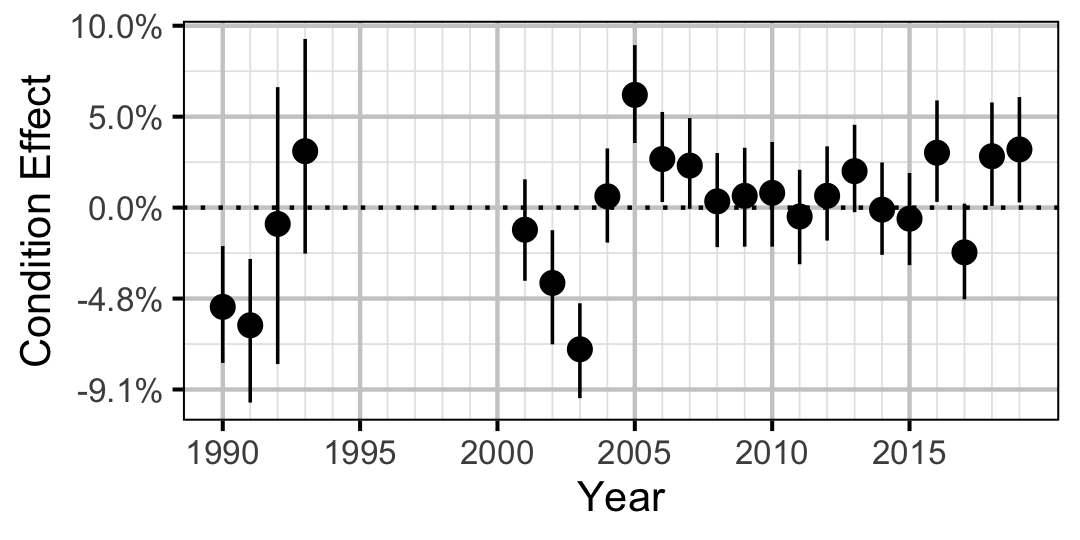Figure 2. Estimated change in condition relative to a typical year for a 200 mm Mountain Whitefish by year (with 95% CRIs).
Rainbow Trout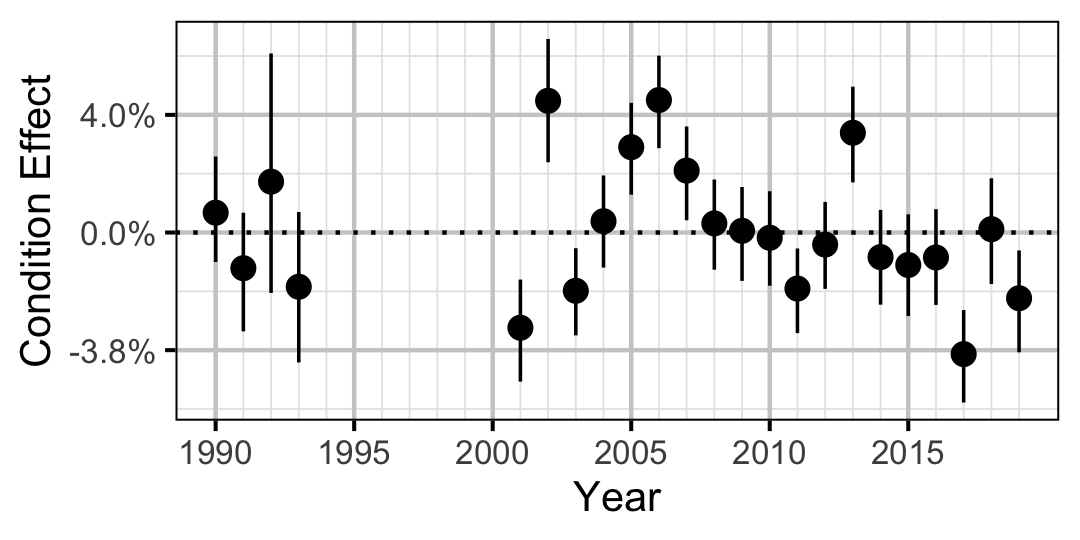Figure 3. Estimated change in condition relative to a typical year for a 250 mm Rainbow Trout by year (with 95% CRIs).
Mountain Whitefish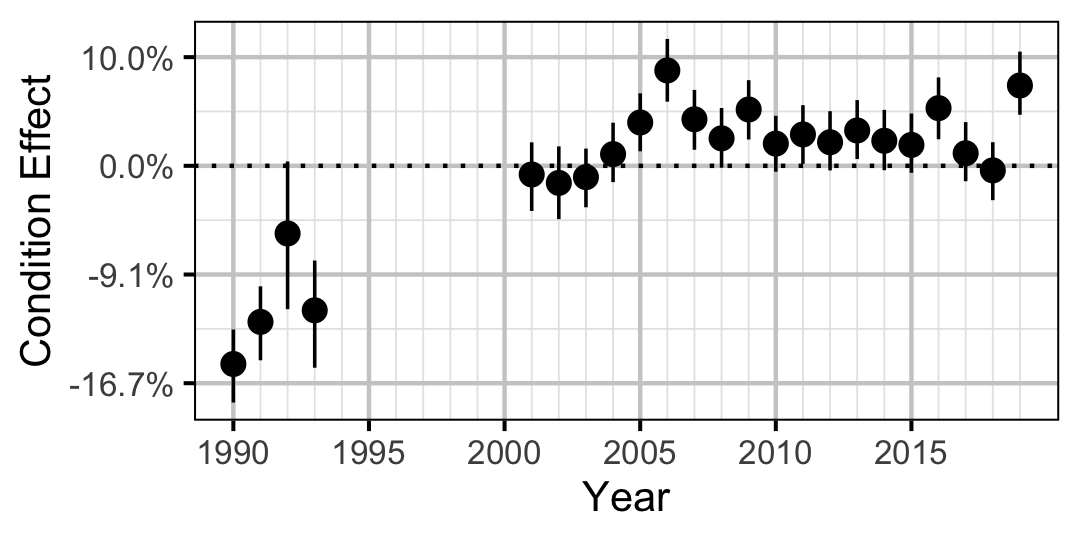Figure 4. Estimated change in condition relative to a typical year for a 350 mm Mountain Whitefish by year (with 95% CRIs).
Rainbow Trout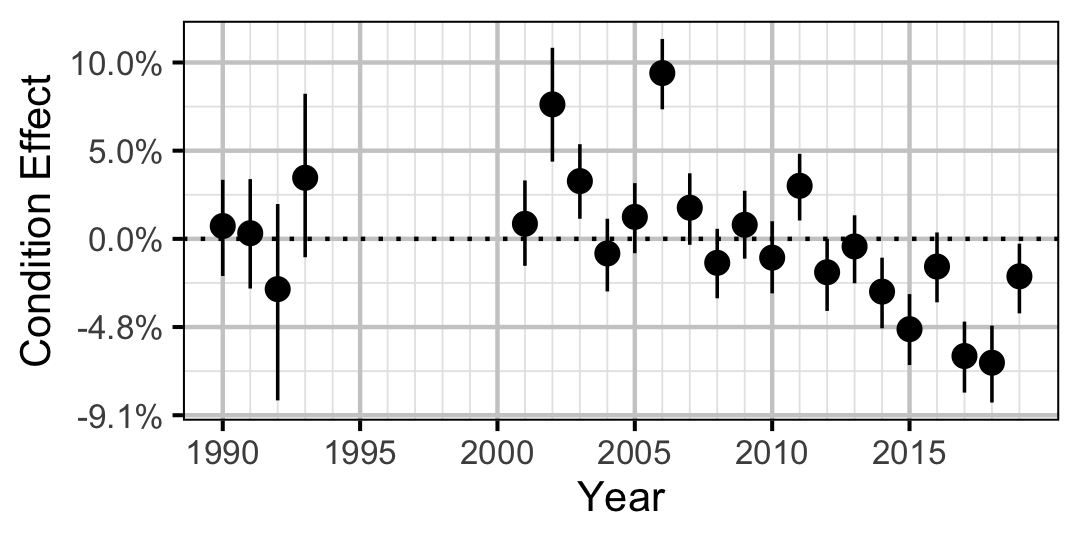Figure 5. Estimated change in condition relative to a typical year for a 500 mm Rainbow Trout by year (with 95% CRIs).
Walleye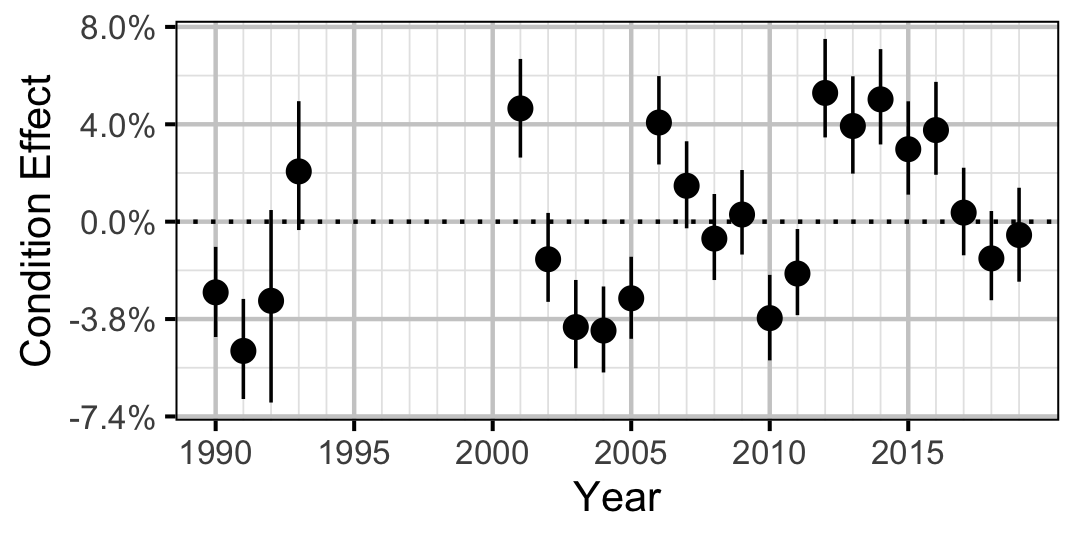Figure 6. Estimated change in condition relative to a typical year for a 400 mm Walleye by year (with 95% CRIs).

Growth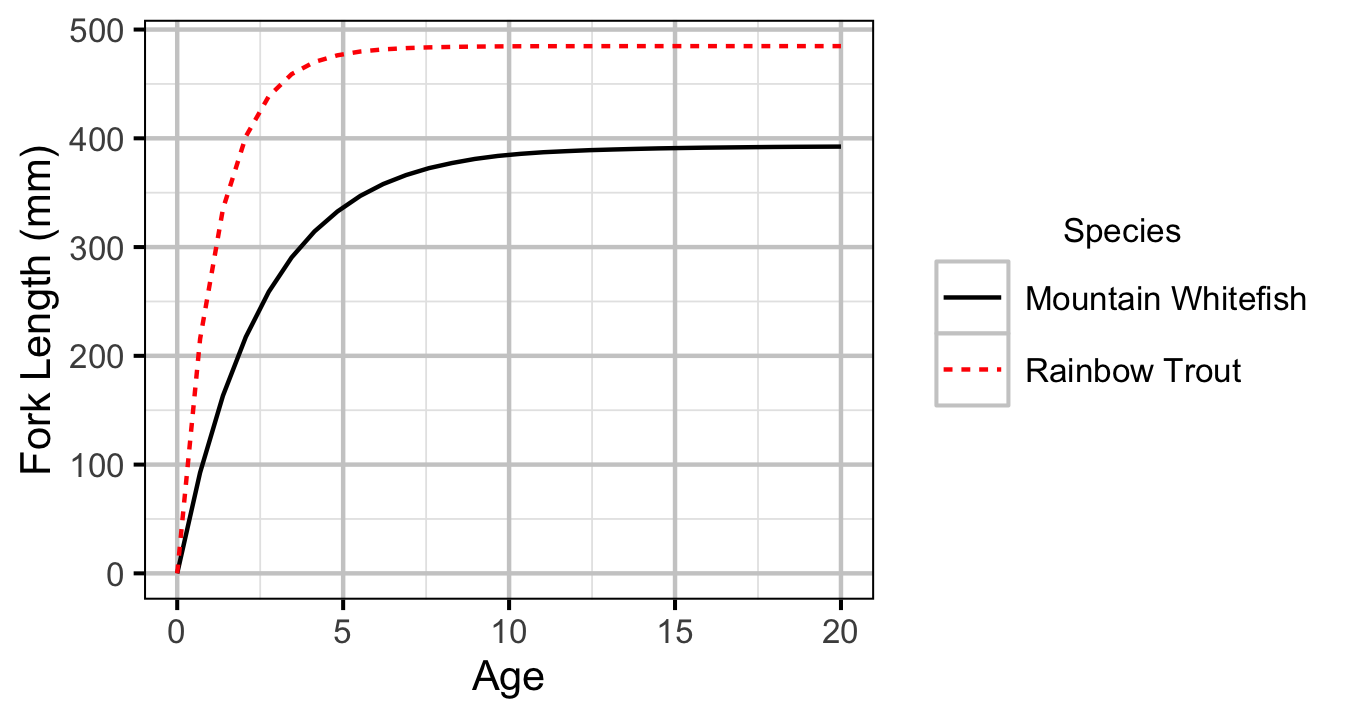Figure 7. Estimated von Bertalanffy growth curve by species. The growth curve for Walleye is not shown because the growth parameters were not representative for the younger age-classes.
Mountain Whitefish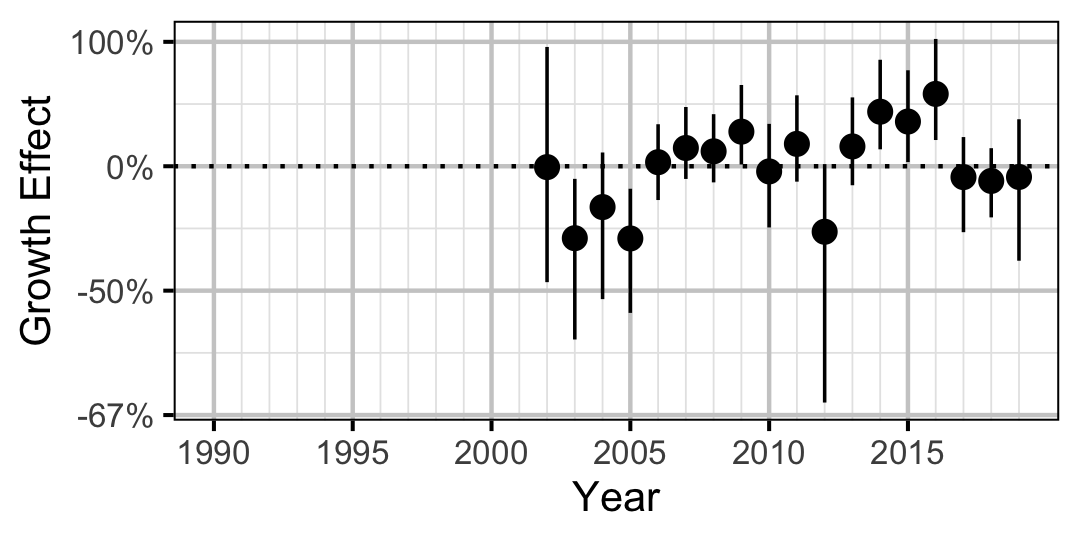Figure 8. Estimated change in von Bertalanffy growth coefficient (k) relative to a typical year by year (with 95% CIs).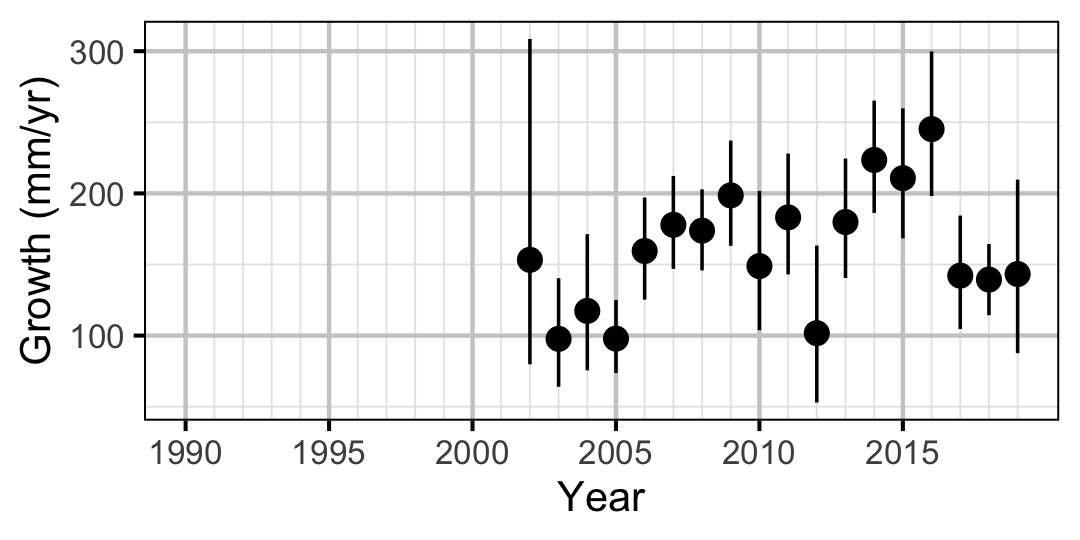Figure 9. Predicted maximum growth by year (with 95% CIs).
Rainbow Trout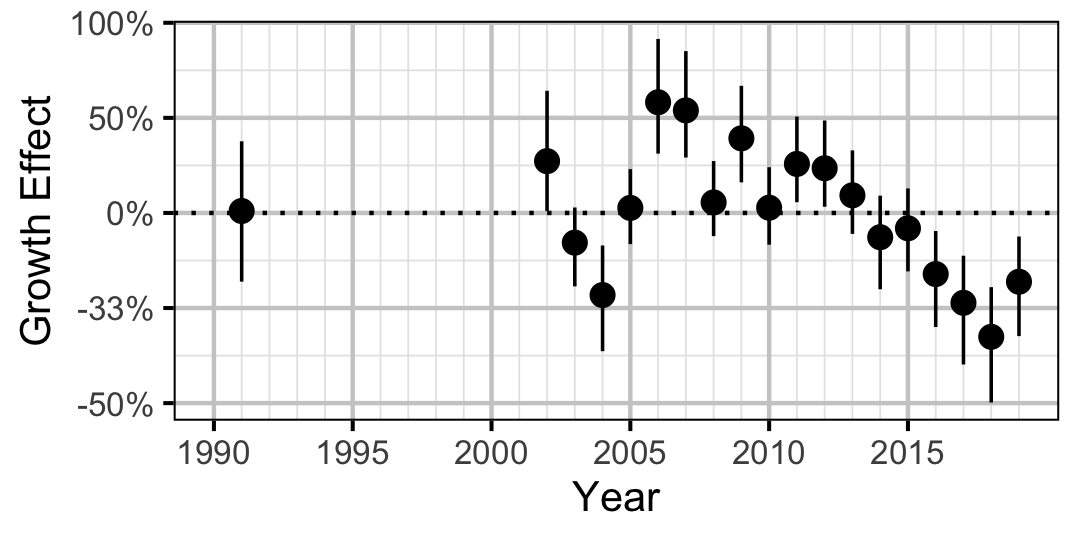Figure 10. Estimated change in von Bertalanffy growth coefficient (k) relative to a typical year by year (with 95% CIs).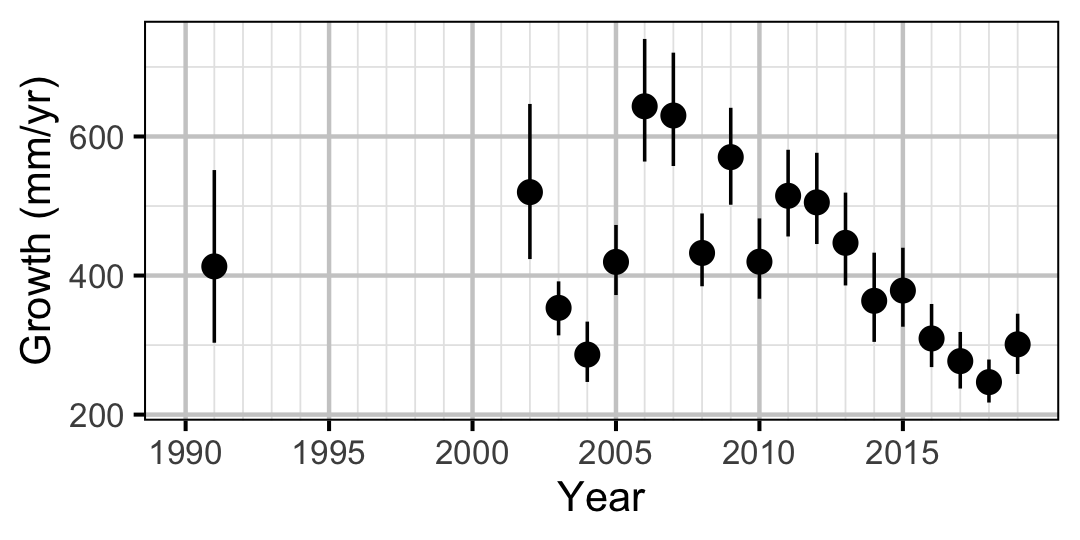Figure 11. Predicted maximum growth by year (with 95% CIs).
Walleye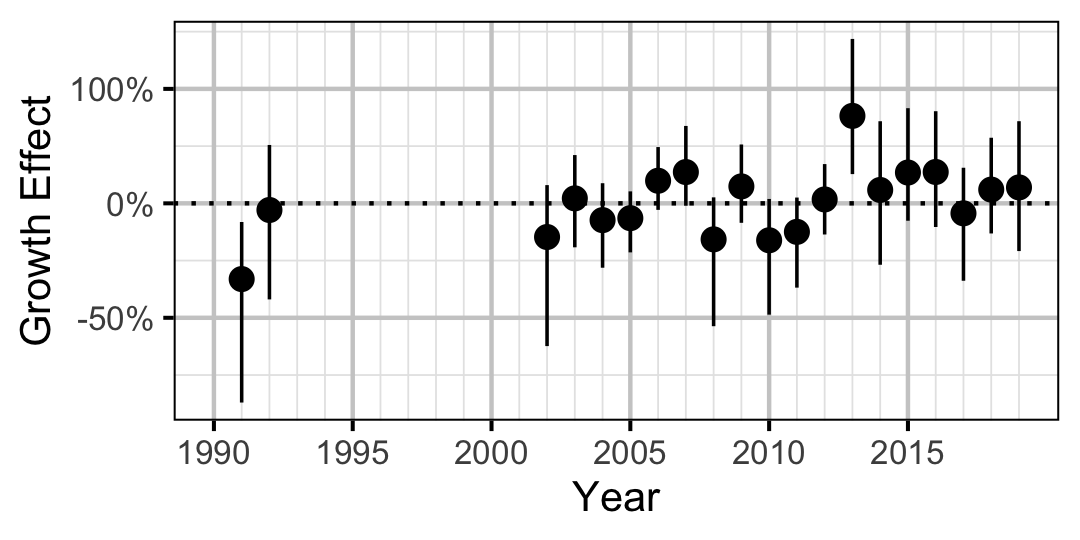Figure 12. Estimated change in von Bertalanffy growth coefficient (k) relative to a typical year by year (with 95% CIs).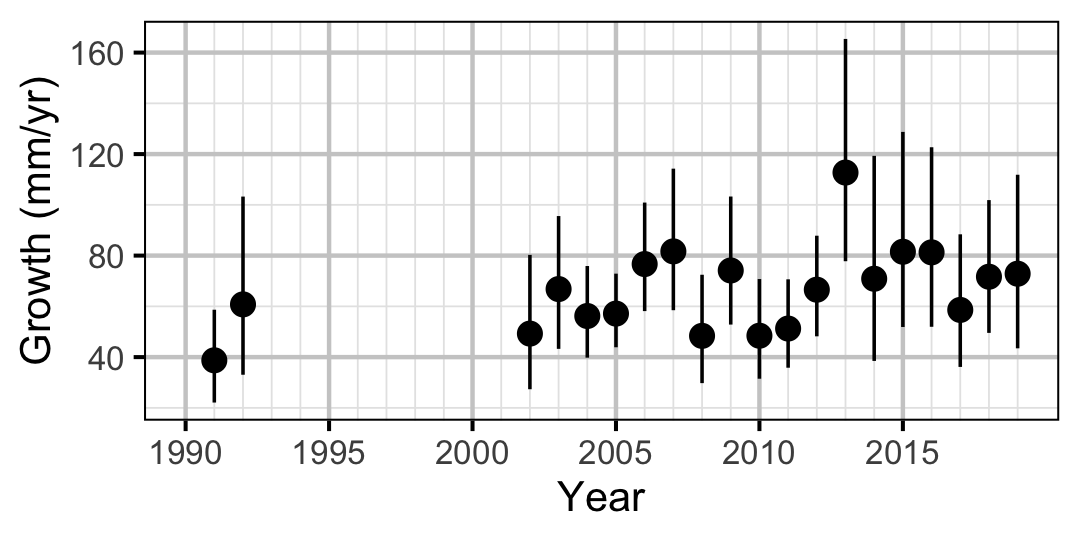Figure 13. Predicted maximum growth by year (with 95% CIs).

Movement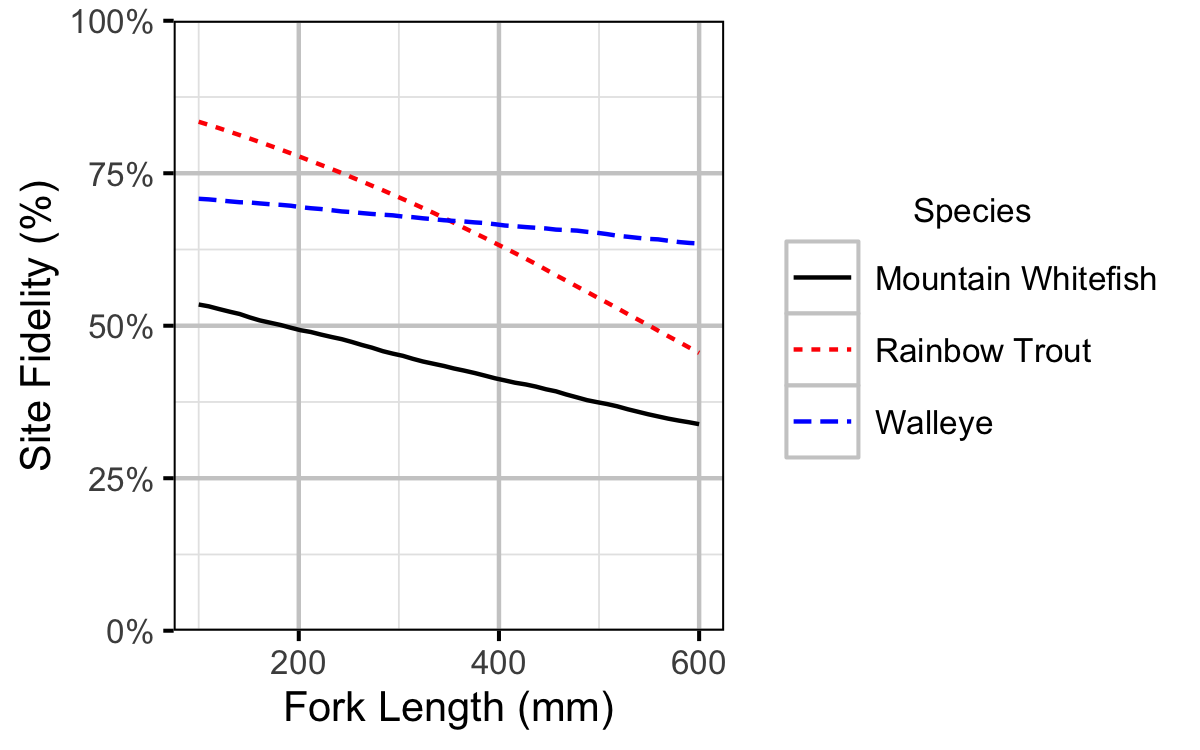Figure 14. Probability of recapture at the same site versus a different site by fish length (with 95% CRIs).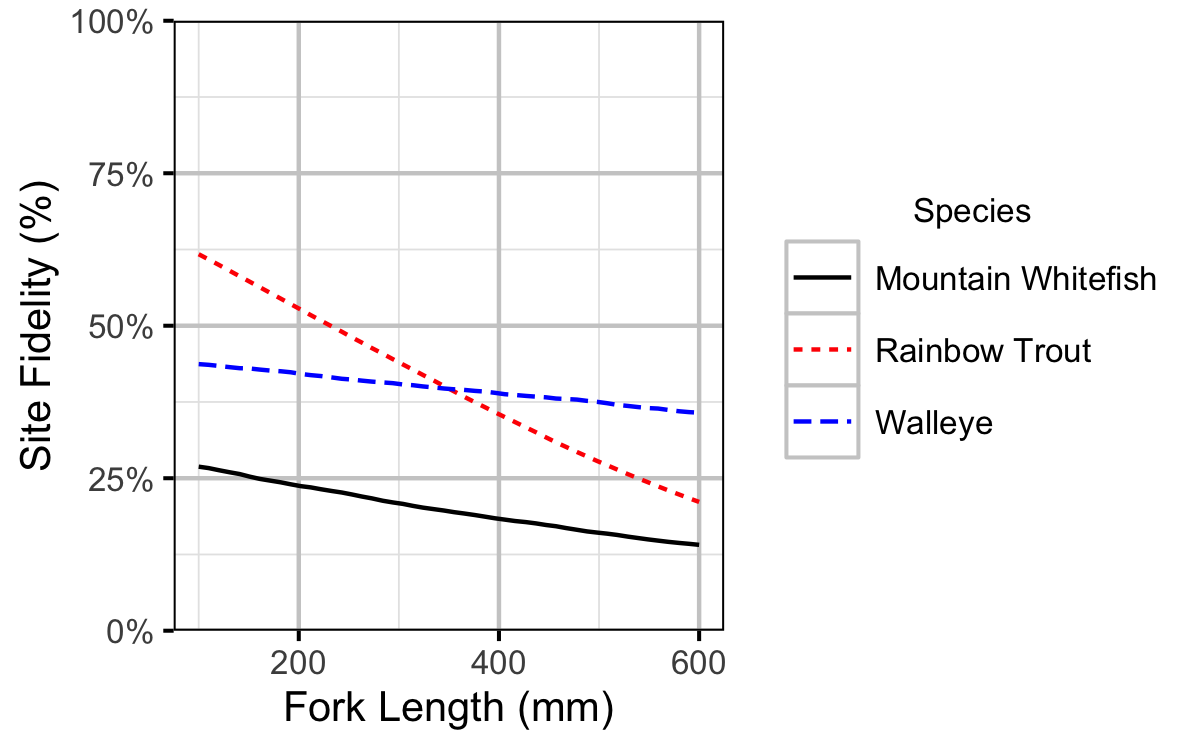Figure 15. Site fidelity by fish length (with 95% CRIs).

Observer Length Correction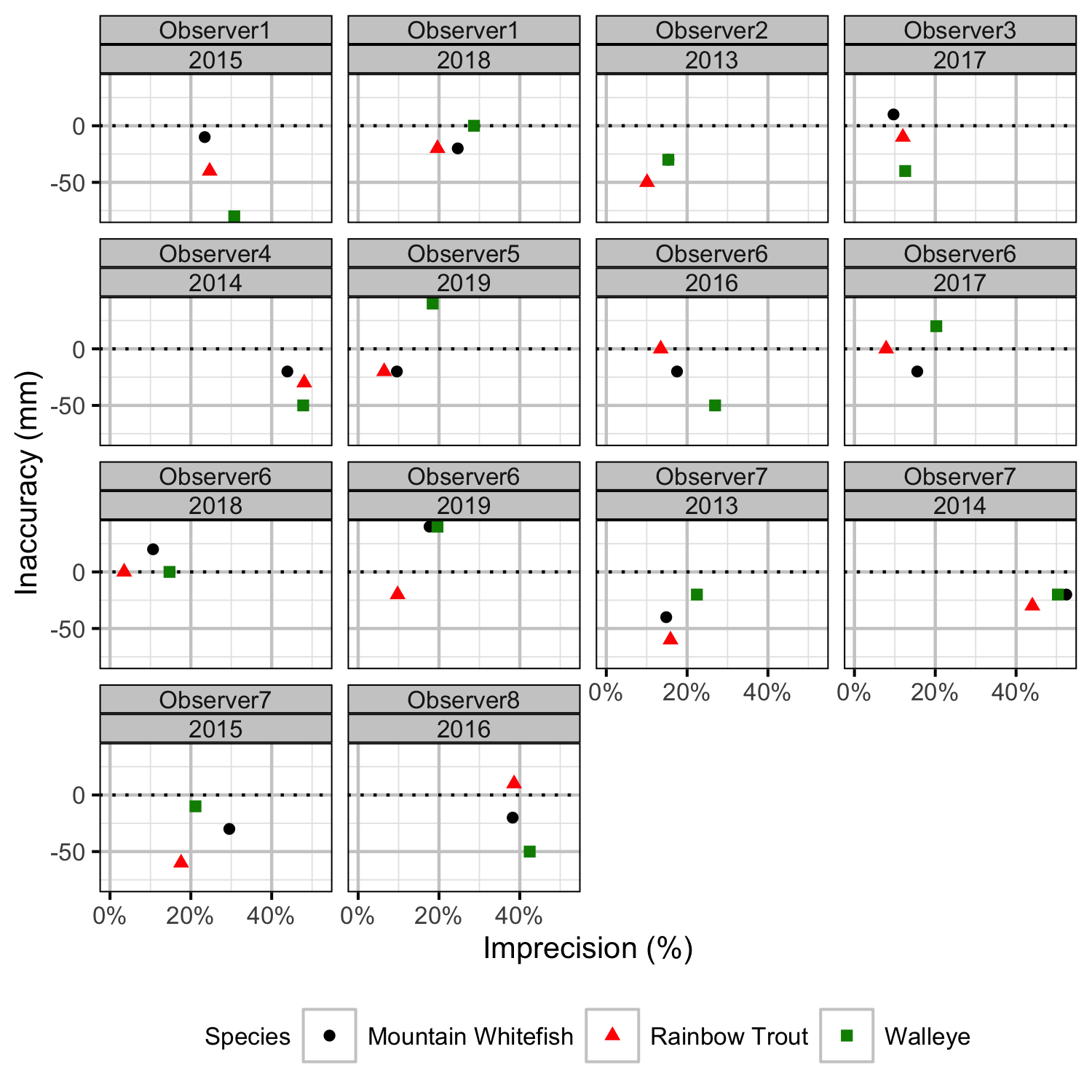Figure 31. Length inaccuracy and imprecision by observer, year and species.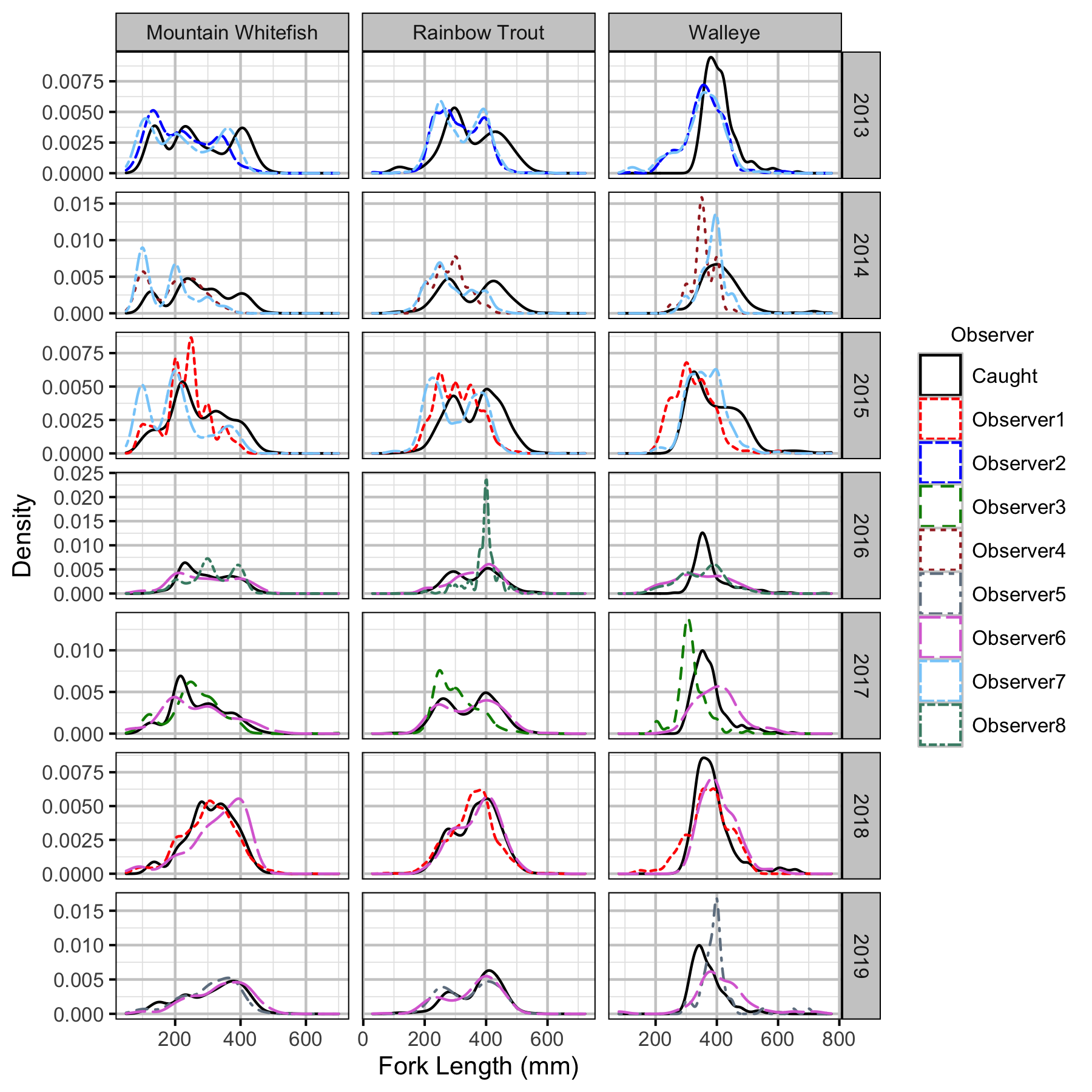Figure 32. Uncorrected length density plots by species, year and observer.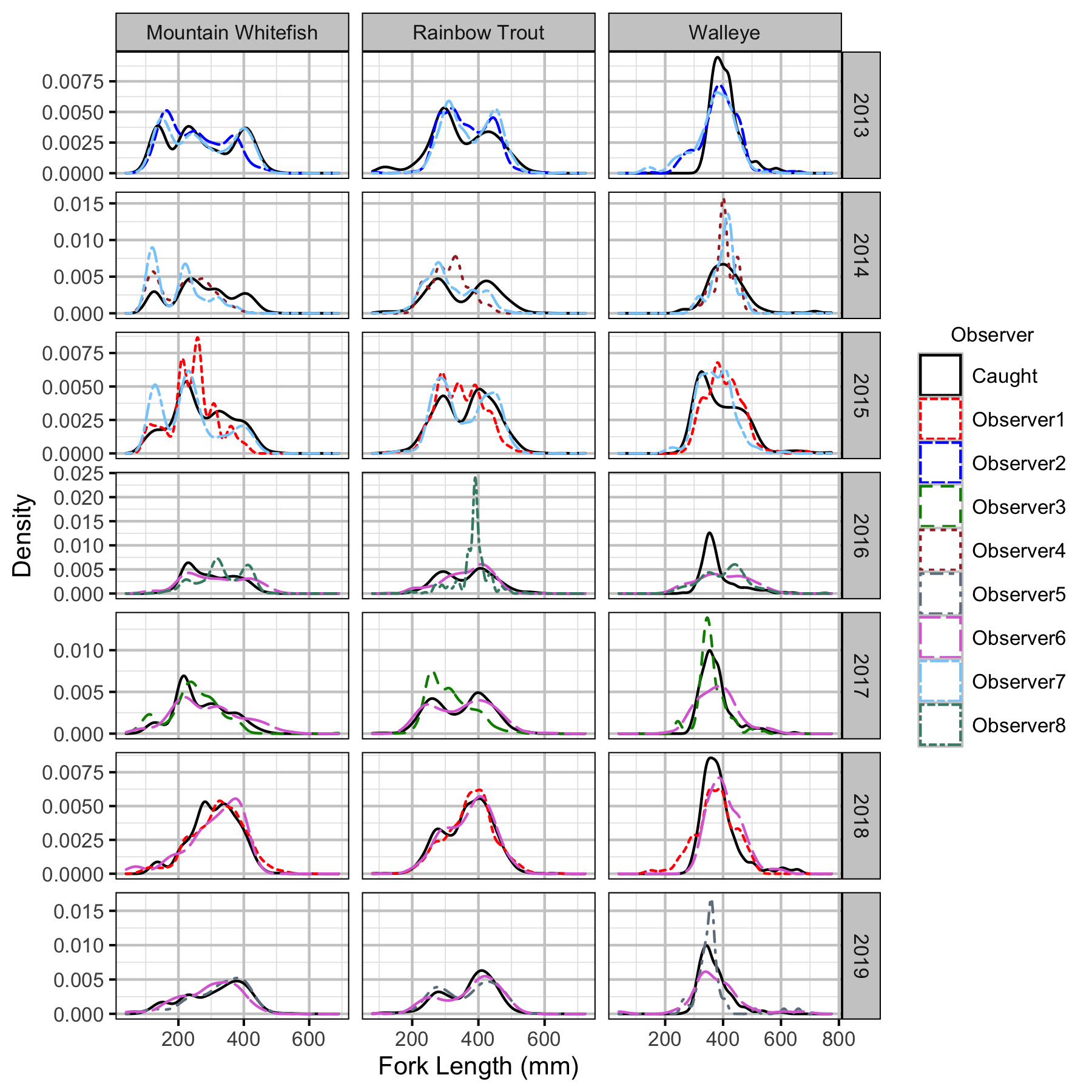Figure 33. Corrected length density plots by species, year and observer.

Capture Efficiency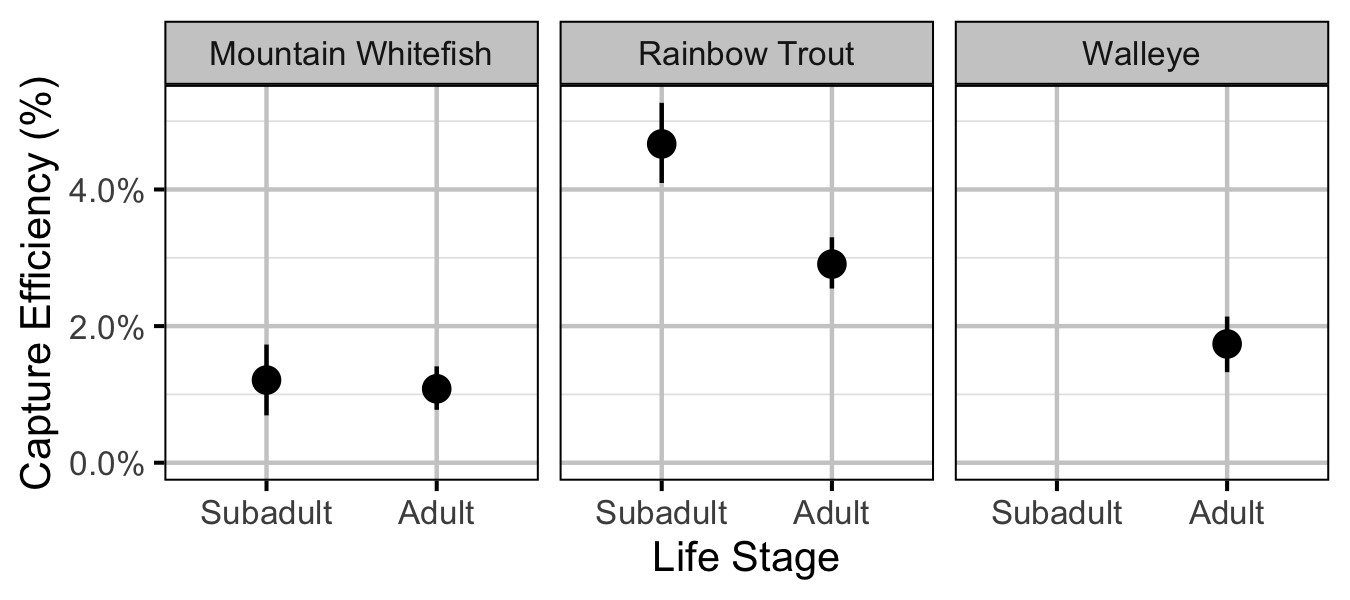Figure 34. Predicted capture efficiency by species and life stage (with 95% CRIs).

Abundance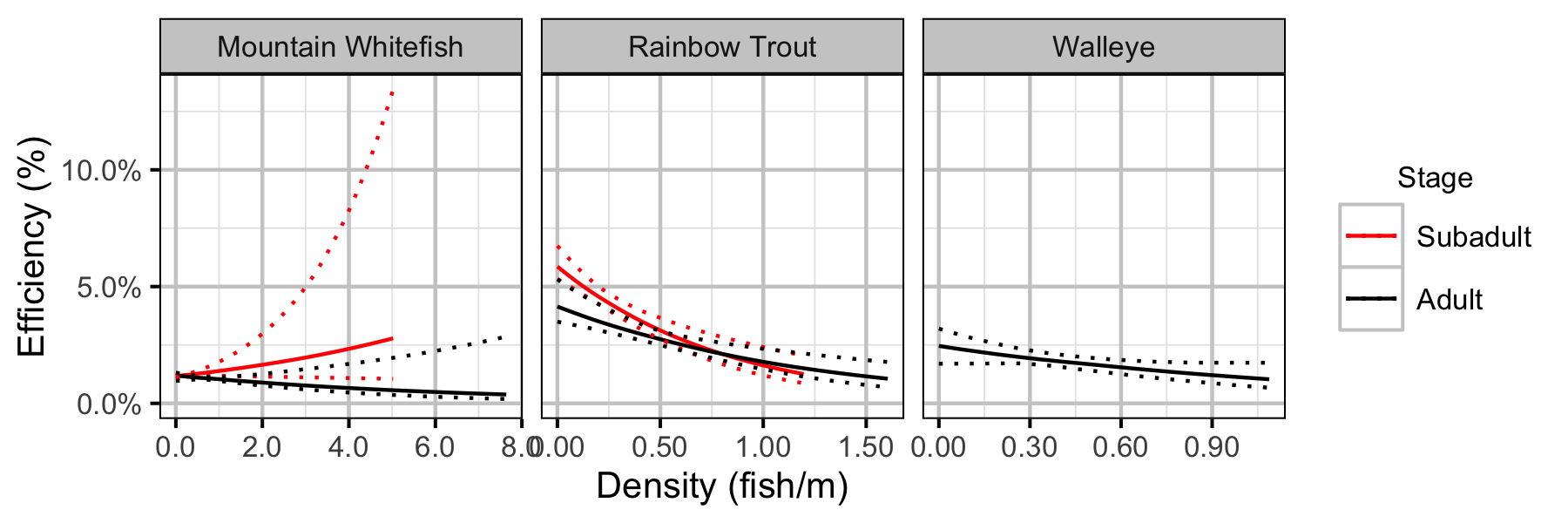Figure 40. Effect of density on capture efficiency by species and stage (with 95% CIs).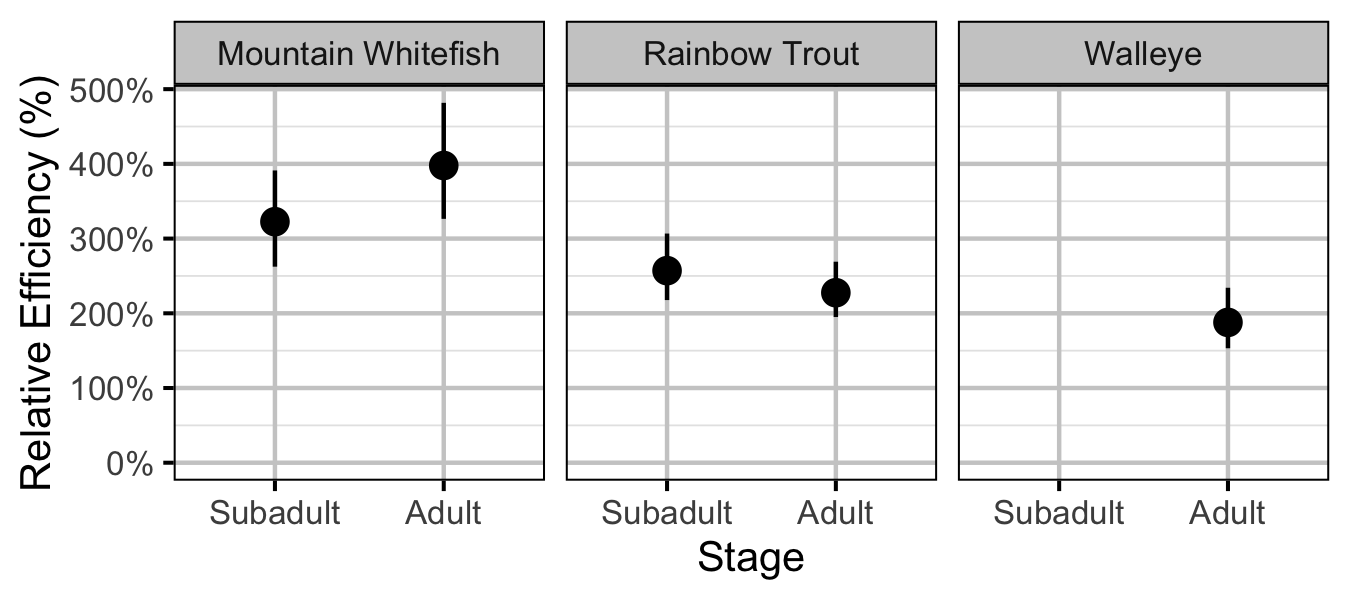Figure 41. Effect of counting (versus capture) on encounter efficiency at typical density by species and stage (with 95% CIs).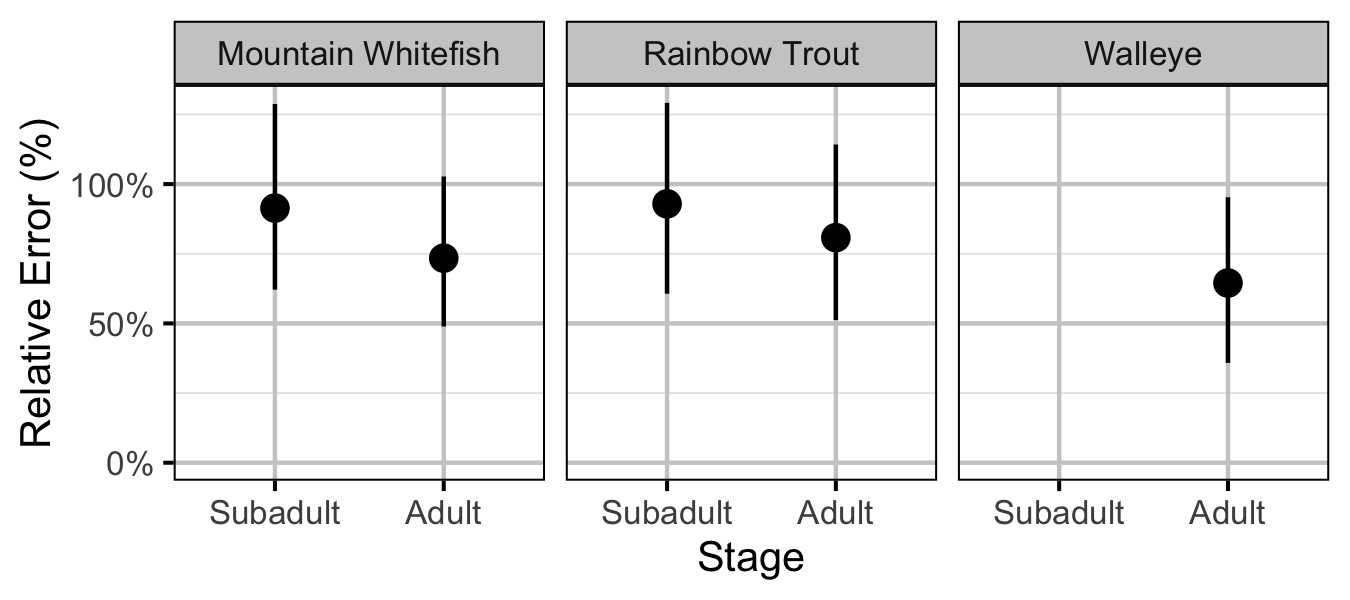Figure 42. Effect of counting (versus capture) on overdispersion by species and stage (with 95% CIs).

Fecundity

Mountain Whitefish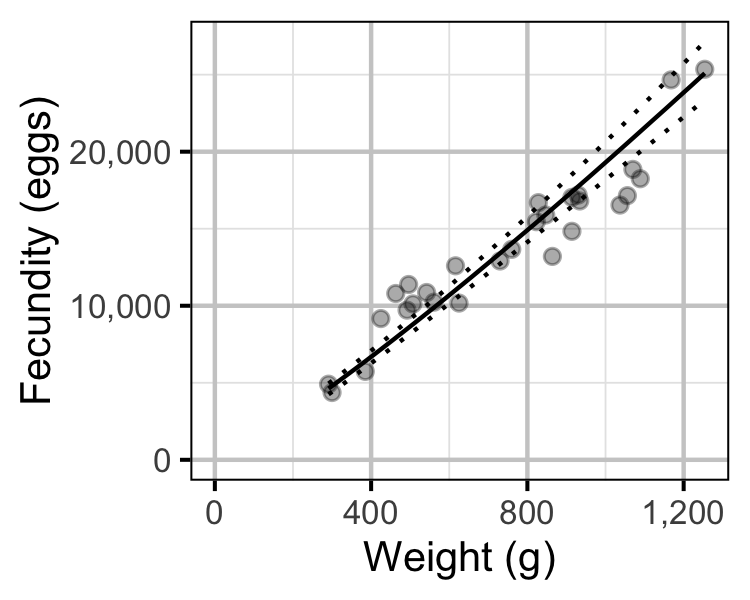Figure 67. The fecundity-weight relationship for Mountain Whitefish (with 95% CRIs). The data are from Boyer et al (2017).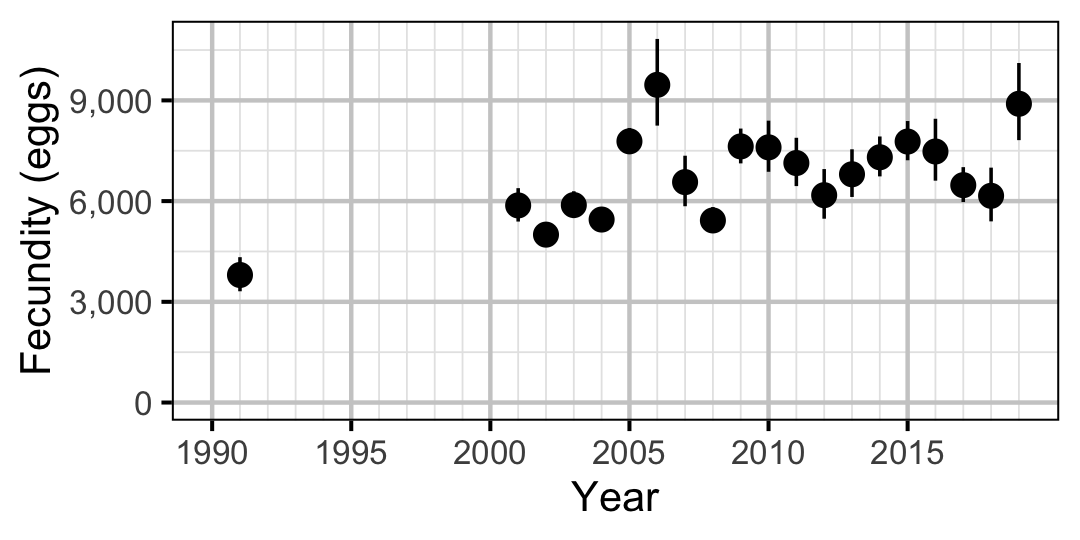Figure 68. Predicted fecundity of an adult female Mountain Whitefish by year (with 95% CIs).

Egg Deposition

Mountain Whitefish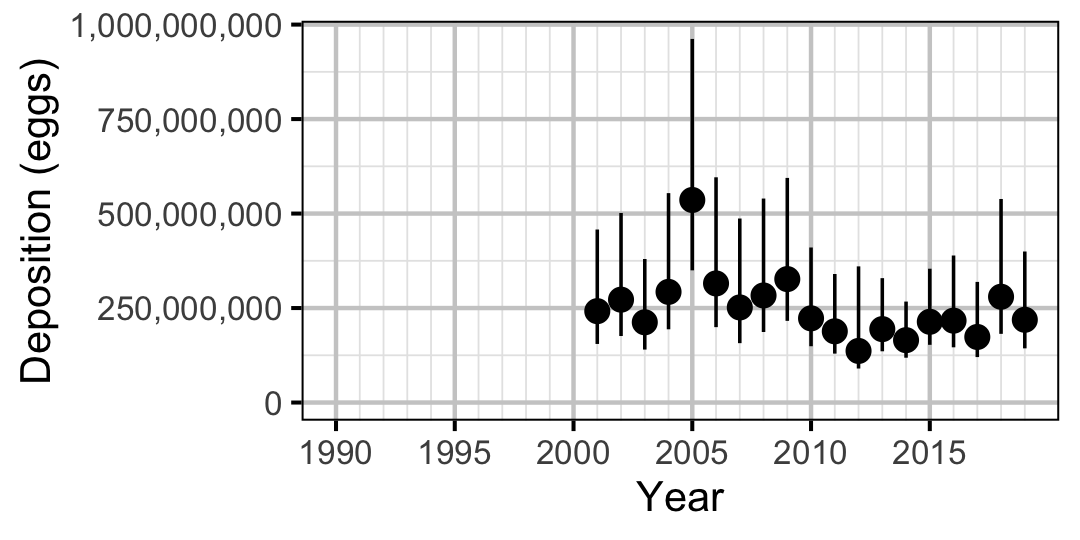Figure 70. Predicted total egg deposition by Mountain Whitefish by year (with 95% CIs).
Rainbow Trout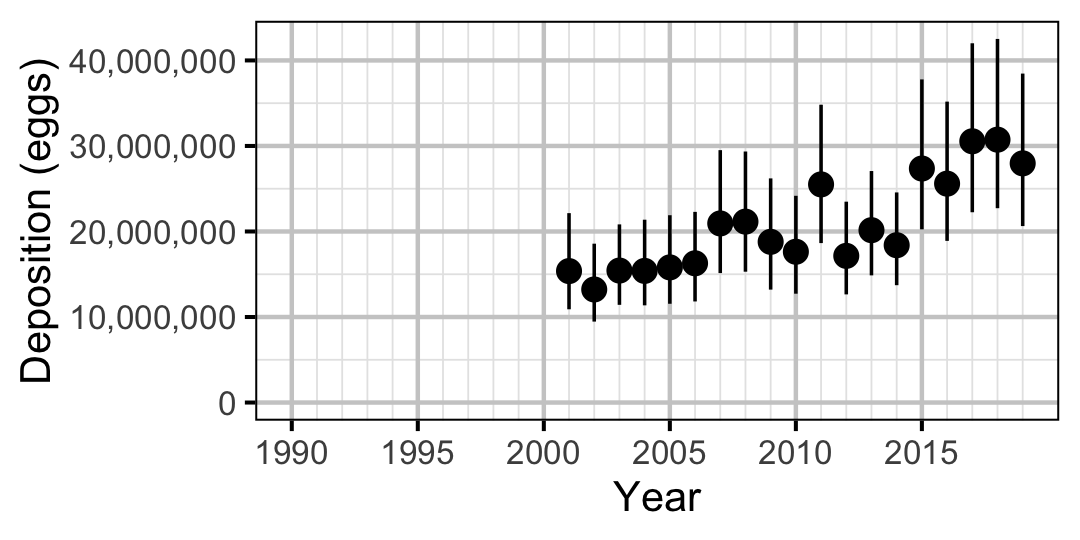Figure 71. Predicted total egg deposition by Rainbow Trout by year (with 95% CIs).

Stock-Recruitment

Mountain Whitefish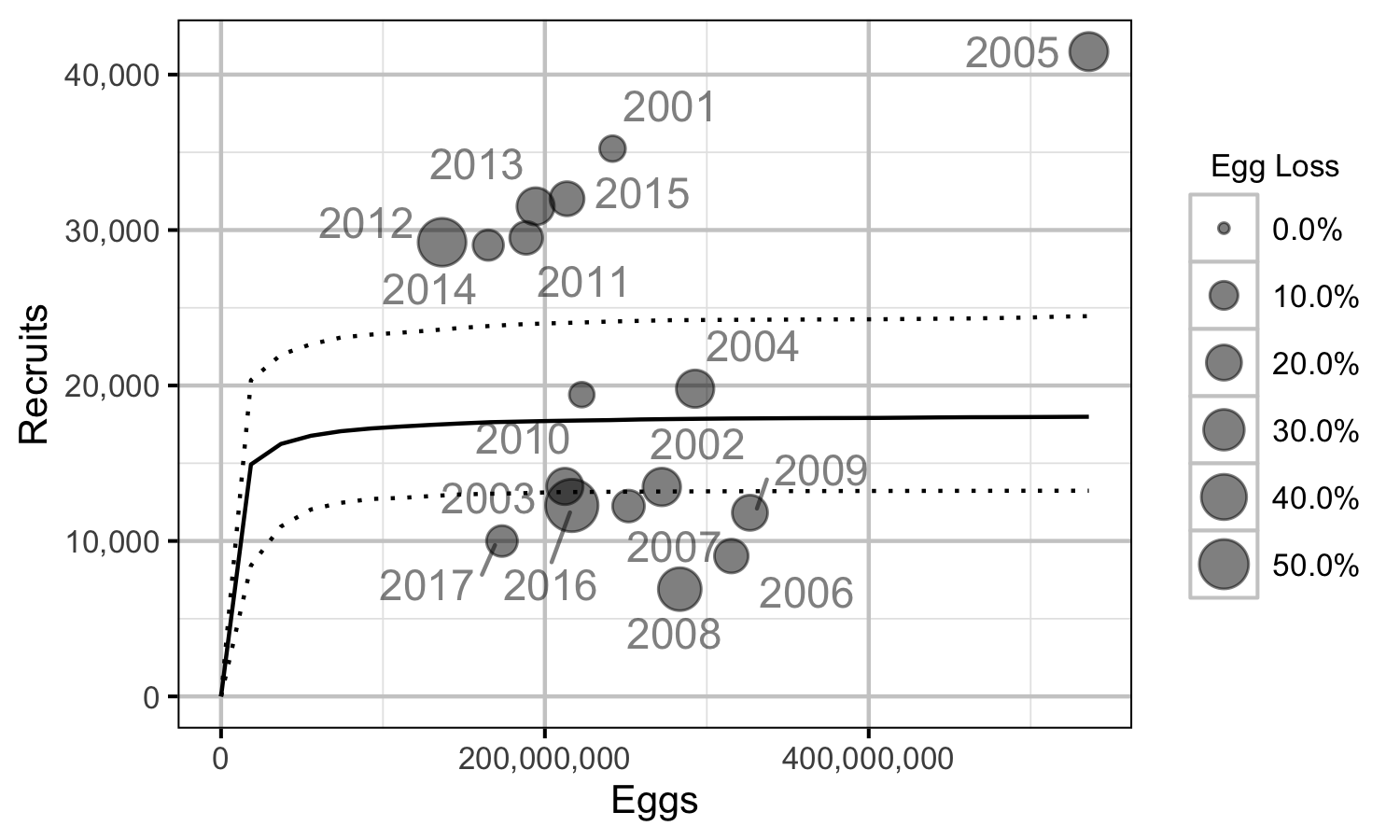Figure 72. Predicted stock-recruitment relationship by spawn year (with 95% CRIs).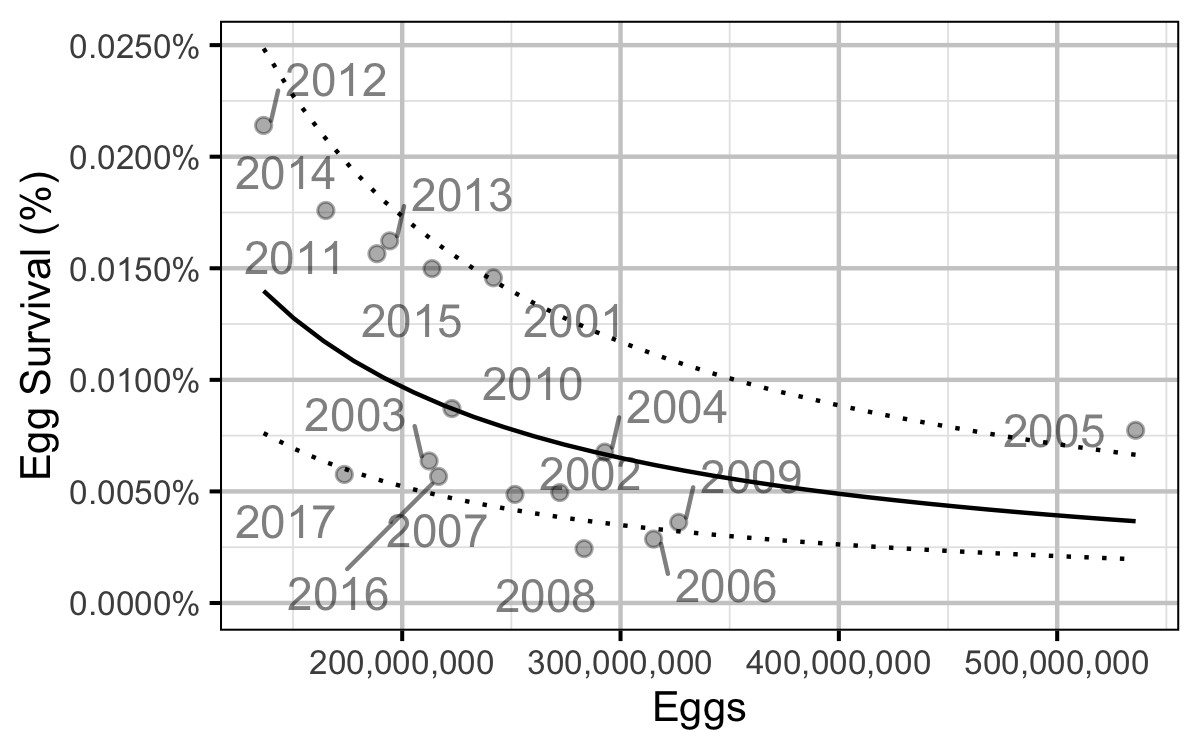Figure 73. Predicted egg to age-1 survival by total egg deposition (with 95% CRIs).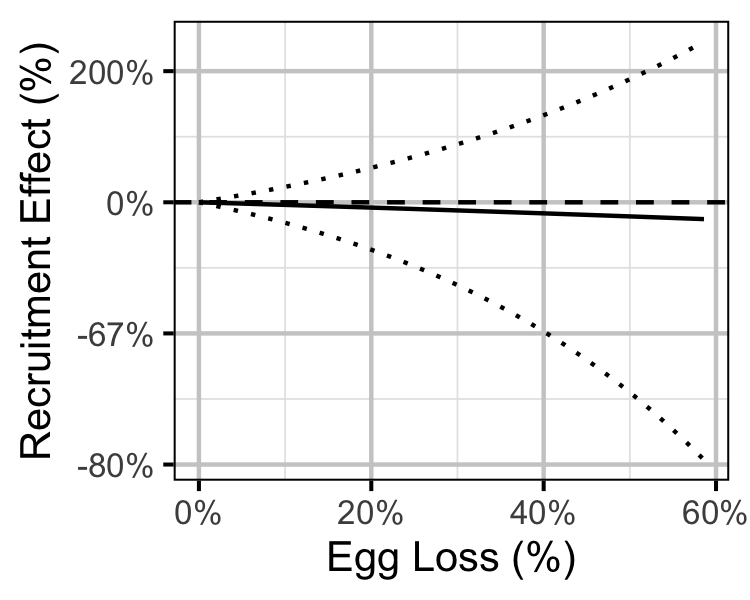Figure 74. Predicted effect of egg loss on the number of age-1 recruits (with 95% CRIs).
Rainbow Trout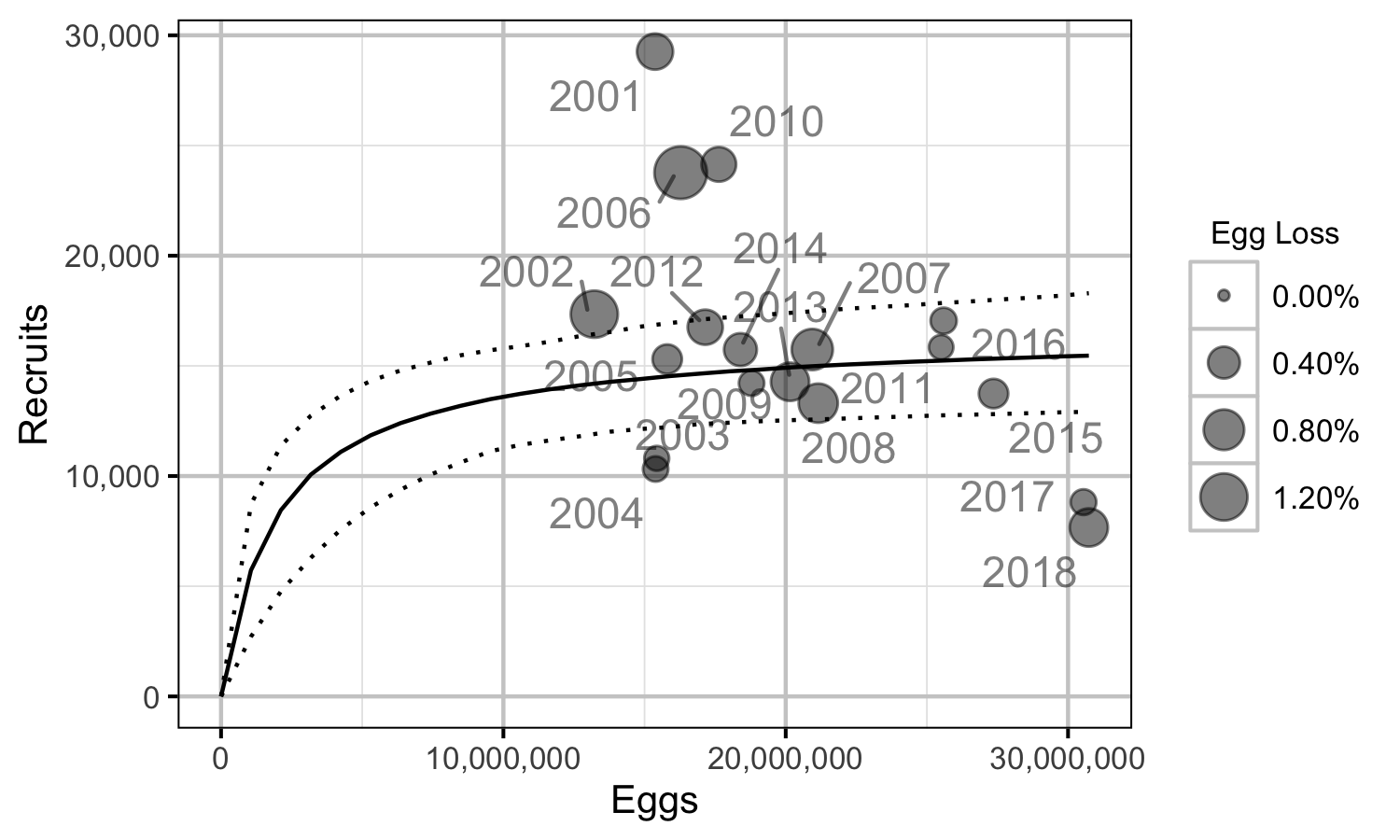Figure 75. Predicted stock-recruitment relationship by spawn year (with 95% CRIs).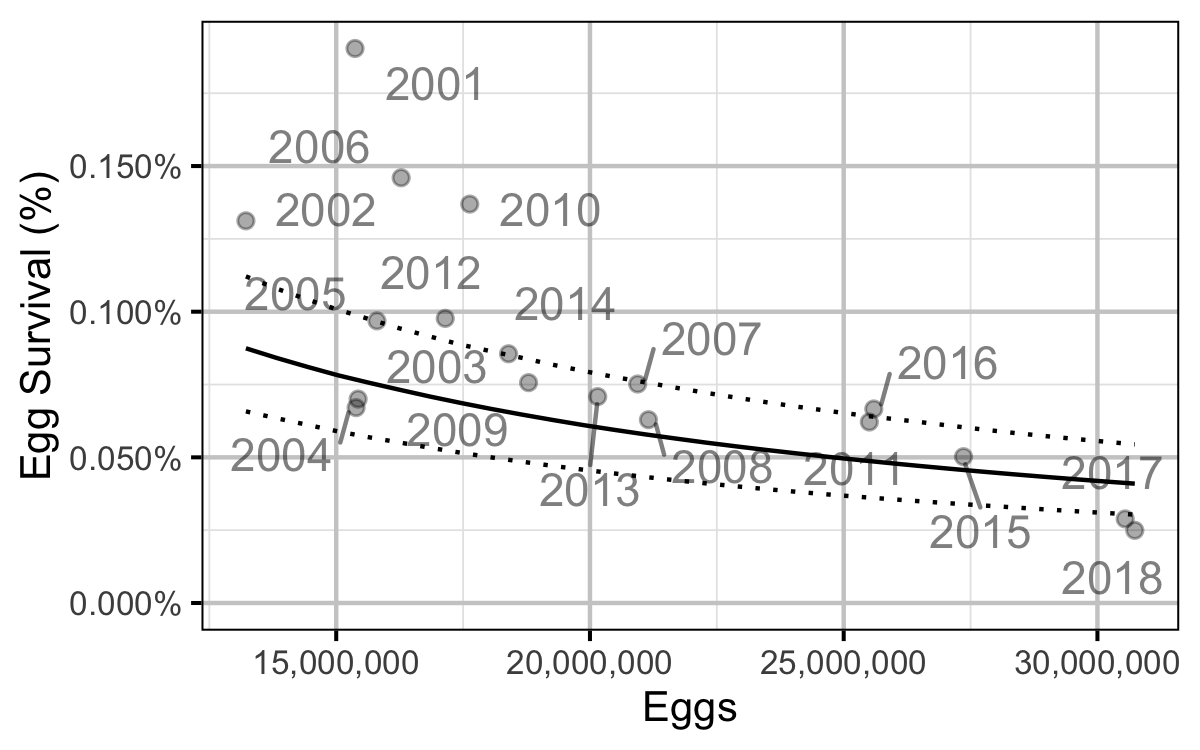Figure 76. Predicted egg to age-1 survival by total egg deposition (with 95% CRIs).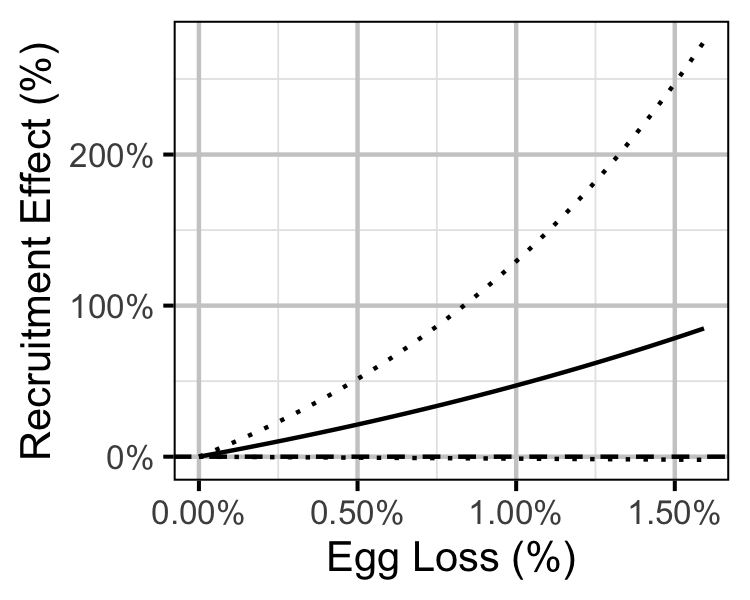Figure 77. Predicted effect of egg loss on the number of age-1 recruits (with 95% CRIs).

Age-Ratios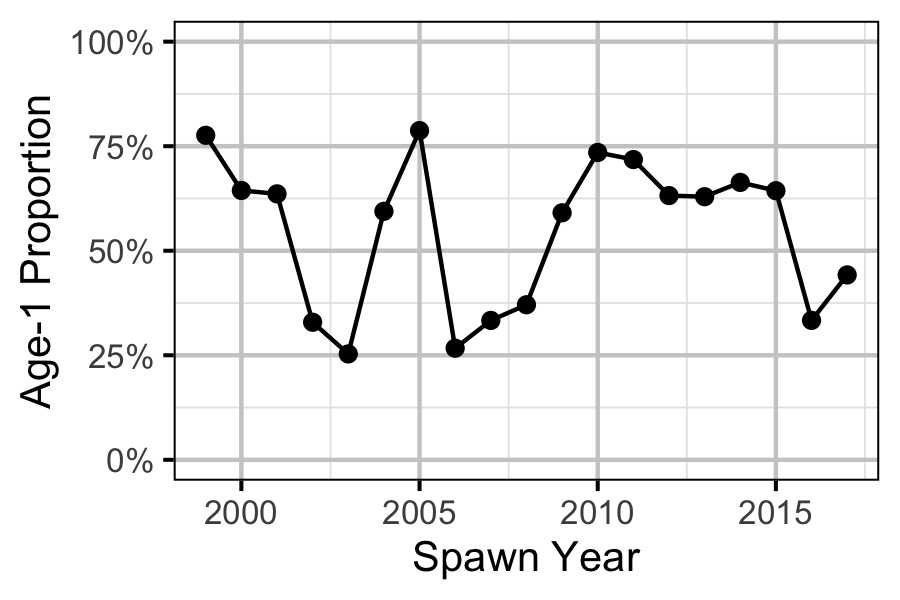Figure 78. Proportion of Age-1 Mountain Whitefish by spawn year.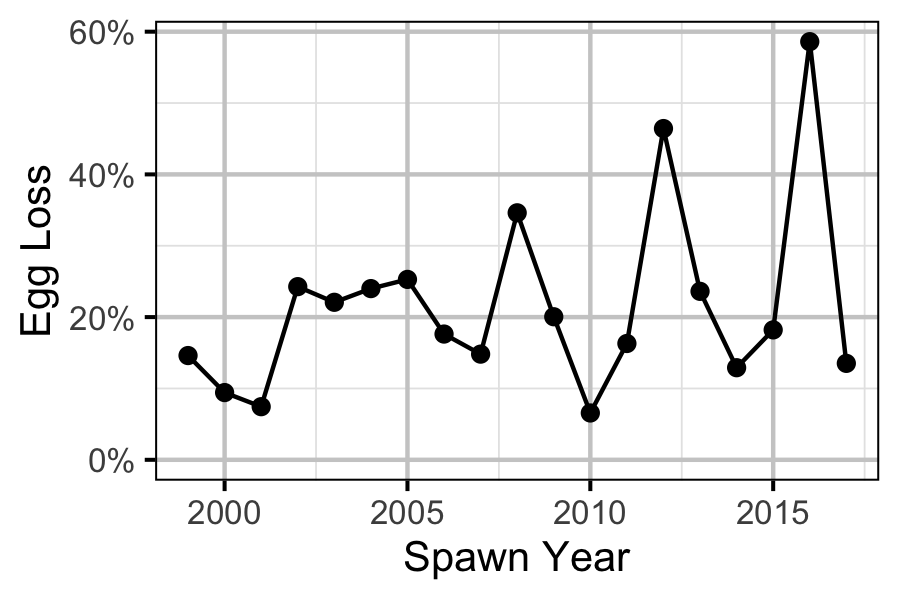Figure 79. Percentage Mountain Whitefish egg loss by spawn year.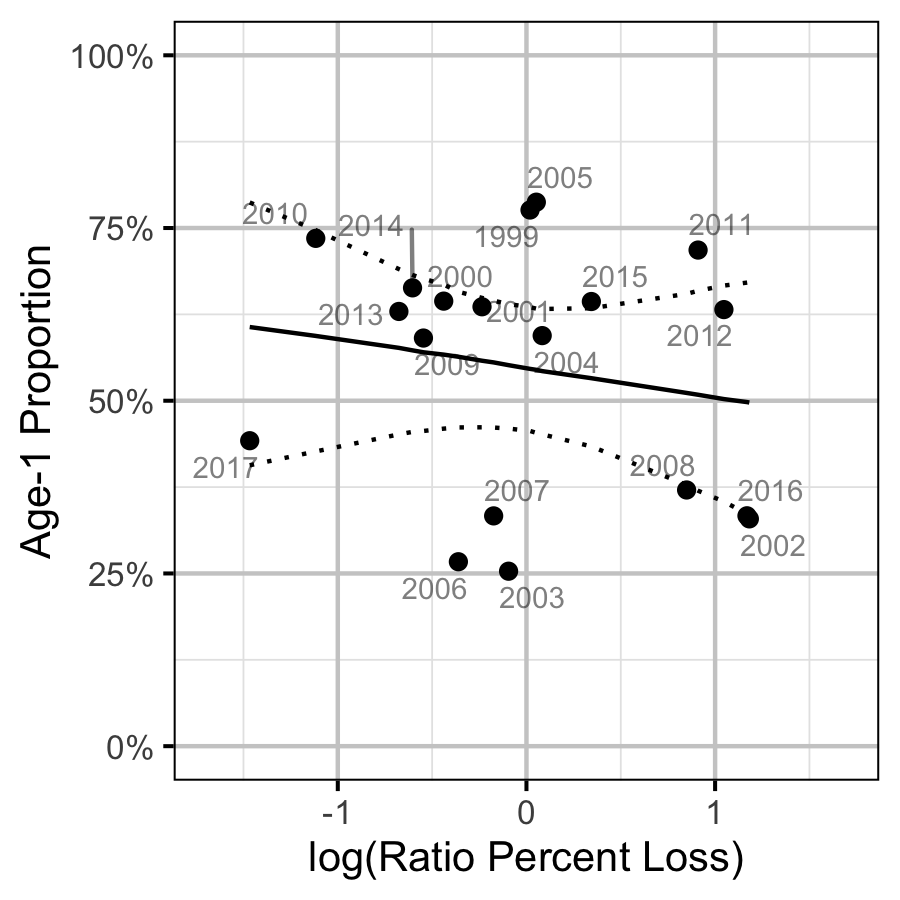Figure 80. Proportion of Age-1 Mountain Whitefish by percentage egg loss ratio, labelled by spawn year. The predicted relationship is indicated by the solid black line (with 95% CRIs).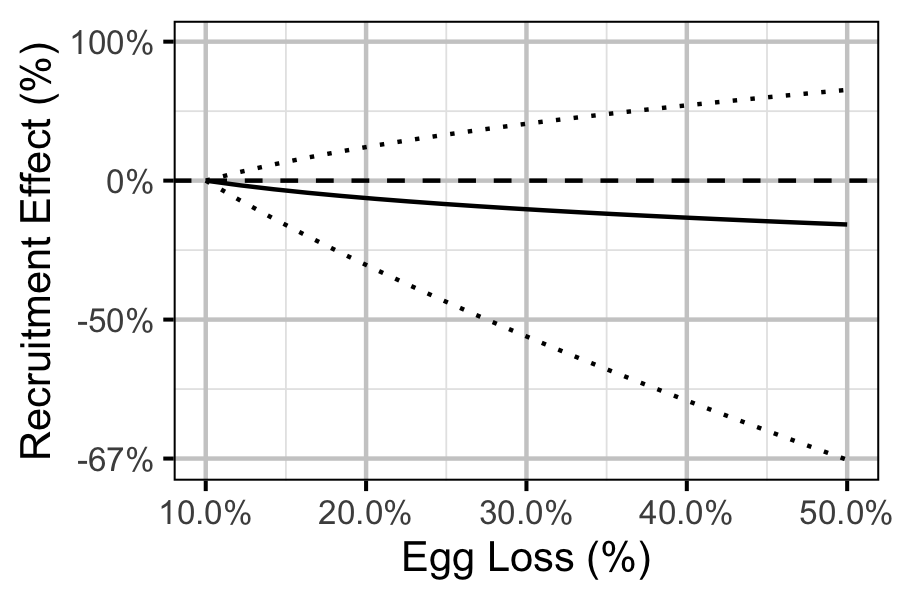Figure 81. Predicted effect of egg loss on the number of age-1 recruits by egg loss relative to 10% egg loss (with 95% CRIs).

Recommendations

• Develop fecundity vs weight relationship for Mountain Whitefish and Rainbow Trout on the Lower Columbia River.

Acknowledgements

The organisations and individuals whose contributions have made this analysis report possible include: